Скачать презентацию The Firms and Theory of Production 2010

1aaf174e09f5525ea628c1dd4d56c4cd.ppt

• Количество слайдов: 81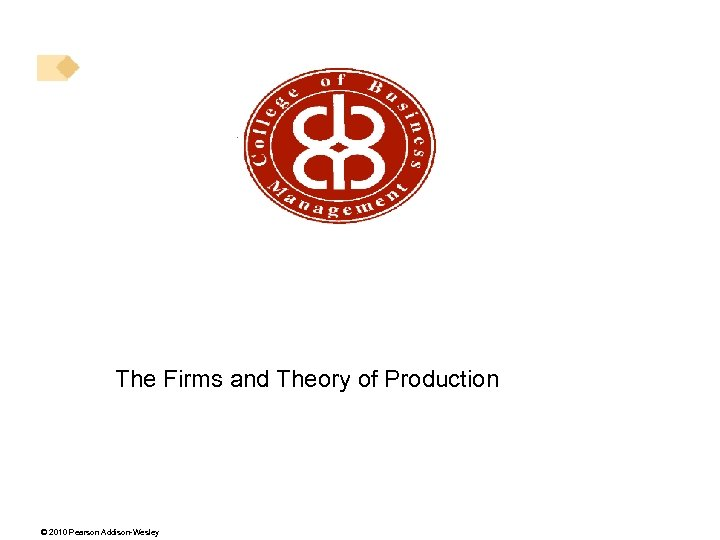The Firms and Theory of Production © 2010 Pearson Addison-Wesley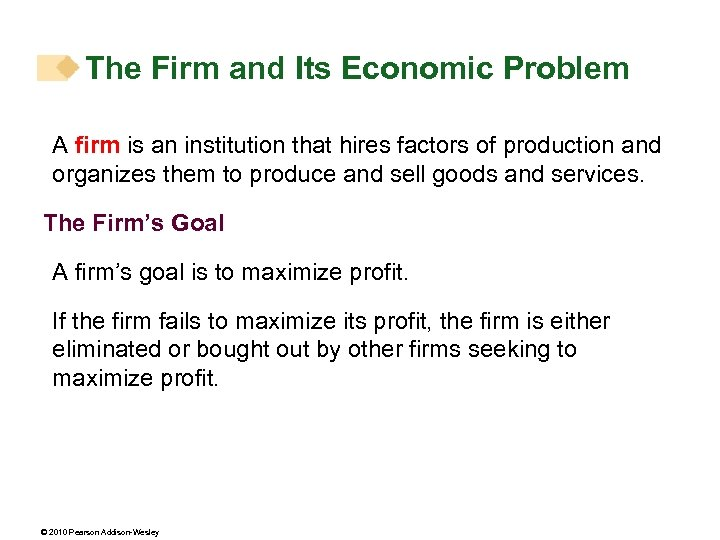The Firm and Its Economic Problem A firm is an institution that hires factors of production and organizes them to produce and sell goods and services. The Firm’s Goal A firm’s goal is to maximize profit. If the firm fails to maximize its profit, the firm is either eliminated or bought out by other firms seeking to maximize profit. © 2010 Pearson Addison-Wesley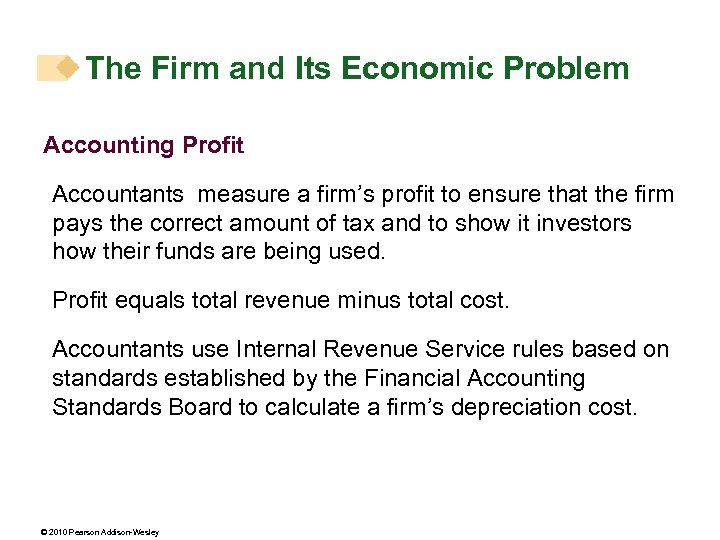The Firm and Its Economic Problem Accounting Profit Accountants measure a firm’s profit to ensure that the firm pays the correct amount of tax and to show it investors how their funds are being used. Profit equals total revenue minus total cost. Accountants use Internal Revenue Service rules based on standards established by the Financial Accounting Standards Board to calculate a firm’s depreciation cost. © 2010 Pearson Addison-Wesley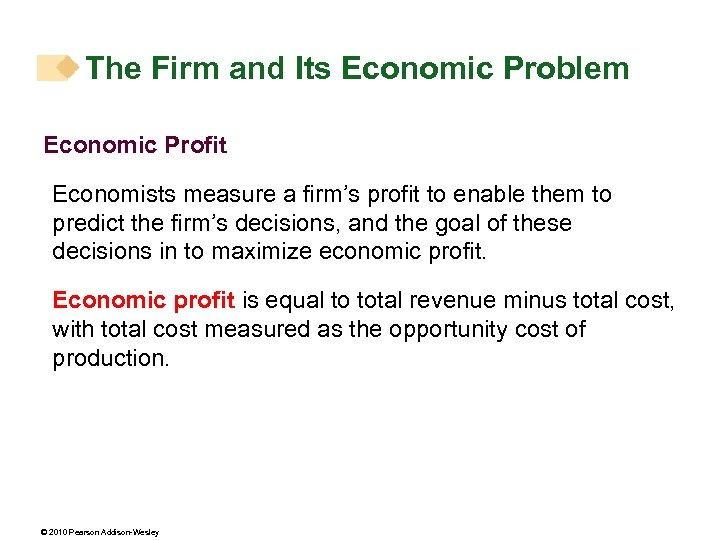The Firm and Its Economic Problem Economic Profit Economists measure a firm’s profit to enable them to predict the firm’s decisions, and the goal of these decisions in to maximize economic profit. Economic profit is equal to total revenue minus total cost, with total cost measured as the opportunity cost of production. © 2010 Pearson Addison-Wesley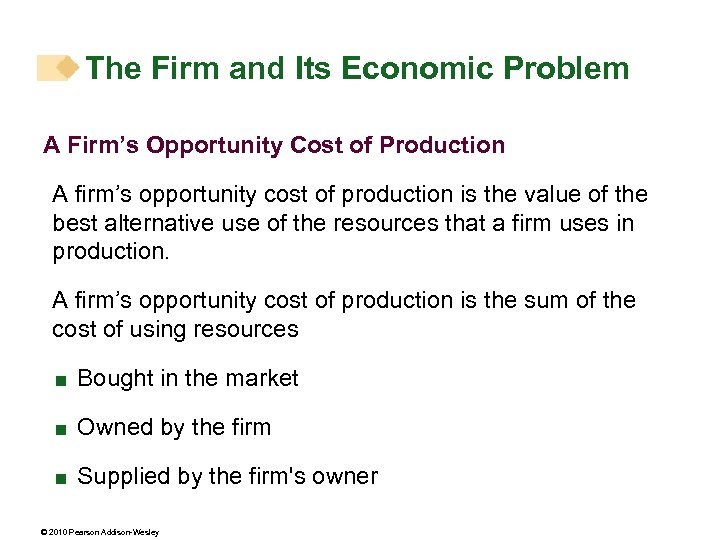The Firm and Its Economic Problem A Firm’s Opportunity Cost of Production A firm’s opportunity cost of production is the value of the best alternative use of the resources that a firm uses in production. A firm’s opportunity cost of production is the sum of the cost of using resources < Bought in the market < Owned by the firm < Supplied by the firm's owner © 2010 Pearson Addison-Wesley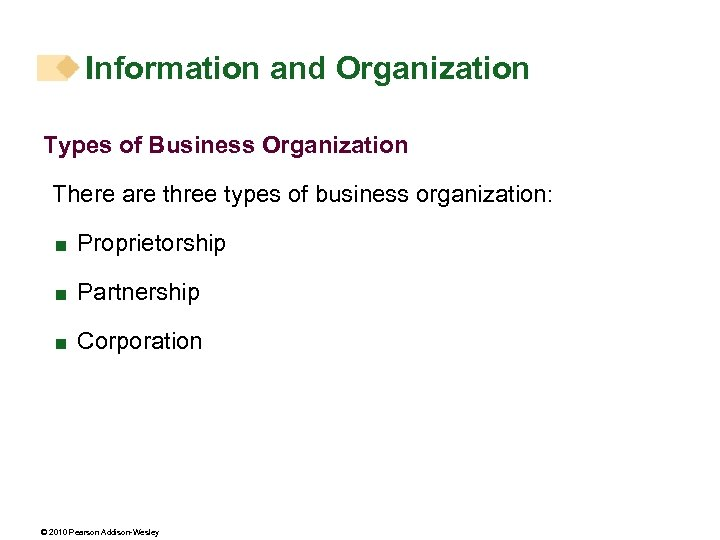Information and Organization Types of Business Organization There are three types of business organization: < Proprietorship < Partnership < Corporation © 2010 Pearson Addison-Wesley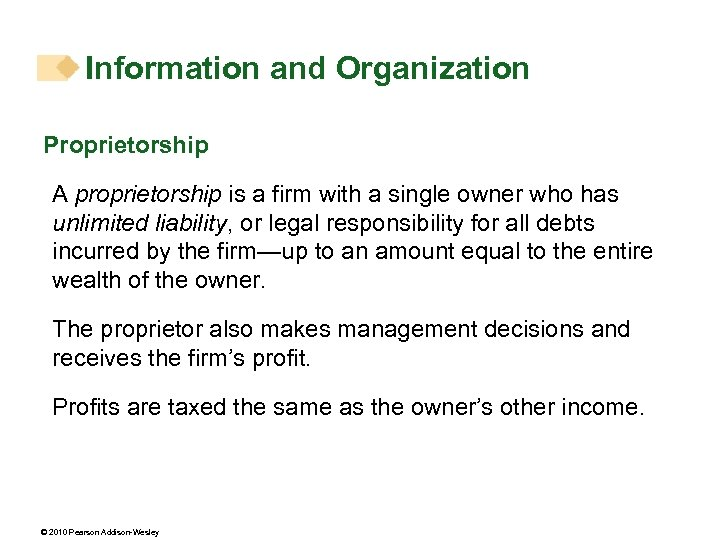Information and Organization Proprietorship A proprietorship is a firm with a single owner who has unlimited liability, or legal responsibility for all debts incurred by the firm—up to an amount equal to the entire wealth of the owner. The proprietor also makes management decisions and receives the firm’s profit. Profits are taxed the same as the owner’s other income. © 2010 Pearson Addison-Wesley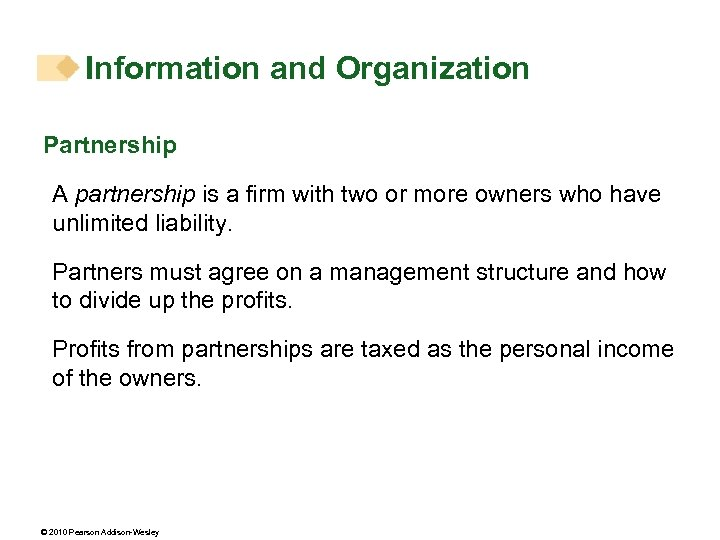Information and Organization Partnership A partnership is a firm with two or more owners who have unlimited liability. Partners must agree on a management structure and how to divide up the profits. Profits from partnerships are taxed as the personal income of the owners. © 2010 Pearson Addison-Wesley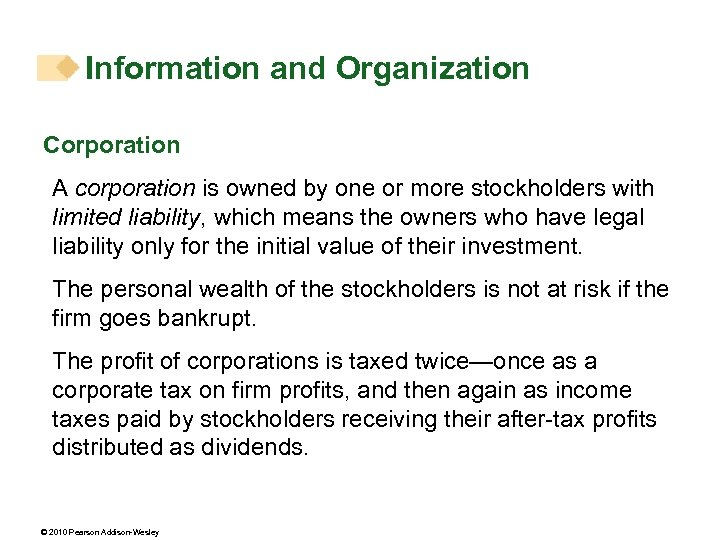Information and Organization Corporation A corporation is owned by one or more stockholders with limited liability, which means the owners who have legal liability only for the initial value of their investment. The personal wealth of the stockholders is not at risk if the firm goes bankrupt. The profit of corporations is taxed twice—once as a corporate tax on firm profits, and then again as income taxes paid by stockholders receiving their after-tax profits distributed as dividends. © 2010 Pearson Addison-Wesley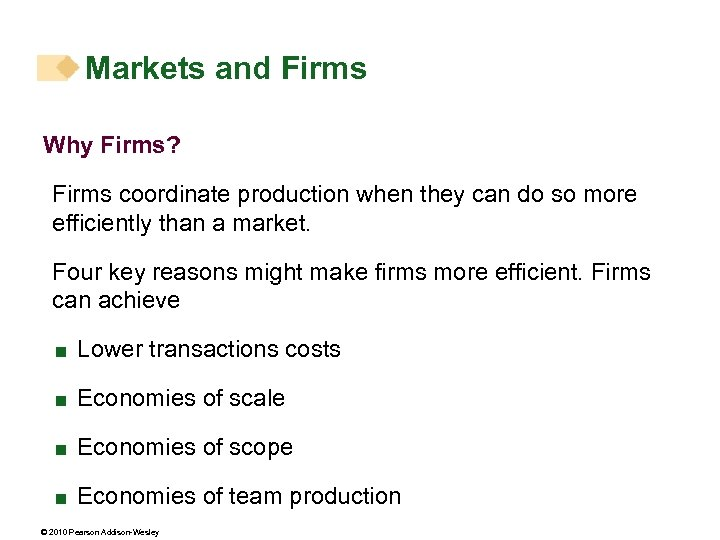Markets and Firms Why Firms? Firms coordinate production when they can do so more efficiently than a market. Four key reasons might make firms more efficient. Firms can achieve < Lower transactions costs < Economies of scale < Economies of scope < Economies of team production © 2010 Pearson Addison-Wesley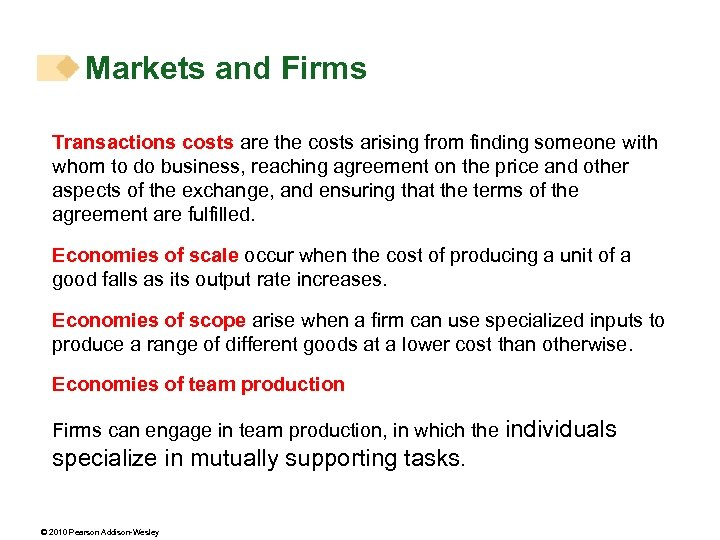Markets and Firms Transactions costs are the costs arising from finding someone with whom to do business, reaching agreement on the price and other aspects of the exchange, and ensuring that the terms of the agreement are fulfilled. Economies of scale occur when the cost of producing a unit of a good falls as its output rate increases. Economies of scope arise when a firm can use specialized inputs to produce a range of different goods at a lower cost than otherwise. Economies of team production Firms can engage in team production, in which the individuals specialize in mutually supporting tasks. © 2010 Pearson Addison-Wesley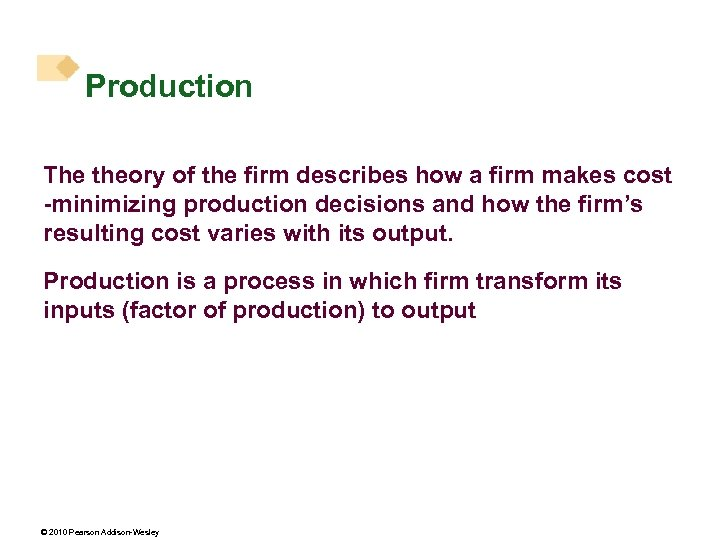Production The theory of the firm describes how a firm makes cost -minimizing production decisions and how the firm’s resulting cost varies with its output. Production is a process in which firm transform its inputs (factor of production) to output © 2010 Pearson Addison-Wesley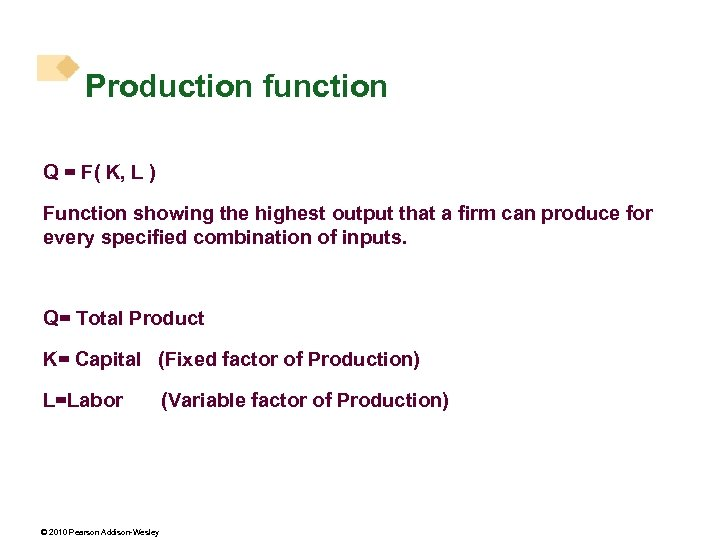Production function Q = F( K, L ) Function showing the highest output that a firm can produce for every specified combination of inputs. Q= Total Product K= Capital (Fixed factor of Production) L=Labor © 2010 Pearson Addison-Wesley (Variable factor of Production)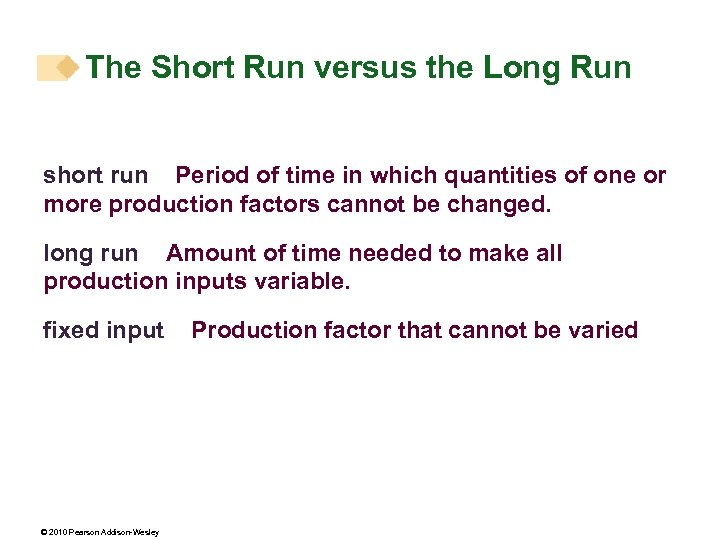The Short Run versus the Long Run short run Period of time in which quantities of one or more production factors cannot be changed. long run Amount of time needed to make all production inputs variable. fixed input © 2010 Pearson Addison-Wesley Production factor that cannot be varied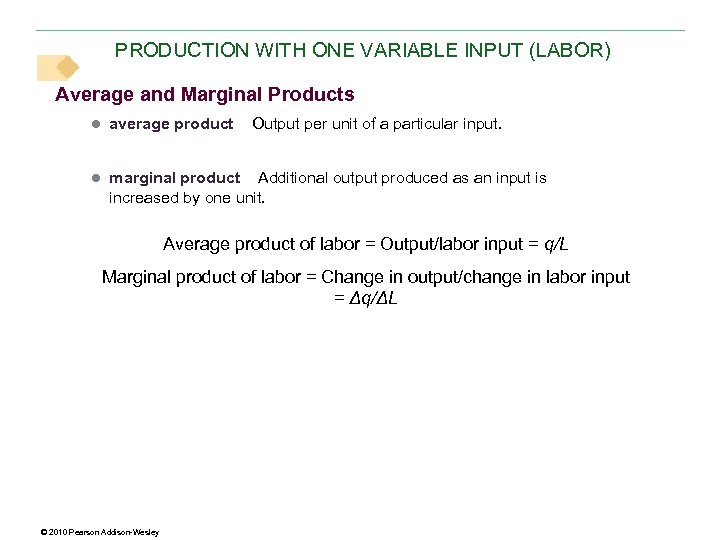PRODUCTION WITH ONE VARIABLE INPUT (LABOR) Average and Marginal Products ● average product Output per unit of a particular input. ● marginal product Additional output produced as an input is increased by one unit. Average product of labor = Output/labor input = q/L Marginal product of labor = Change in output/change in labor input = Δq/ΔL © 2010 Pearson Addison-Wesley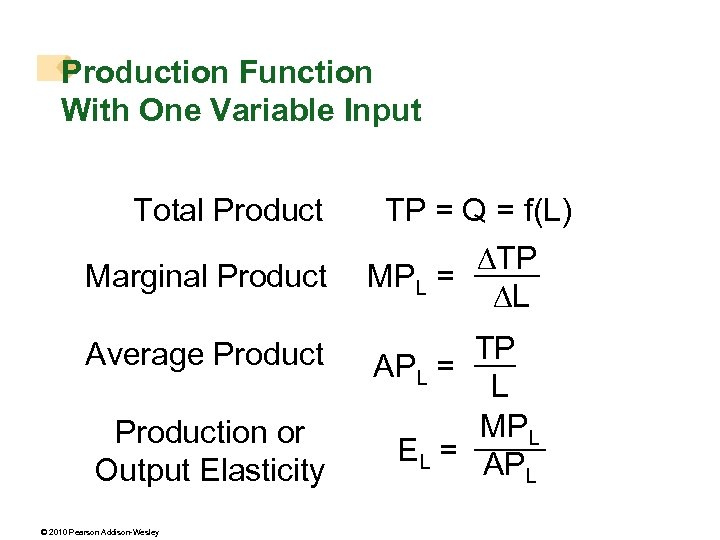Production Function With One Variable Input Total Product Marginal Product Average Production or Output Elasticity © 2010 Pearson Addison-Wesley TP = Q = f(L) TP MPL = L TP APL = L MPL EL = AP L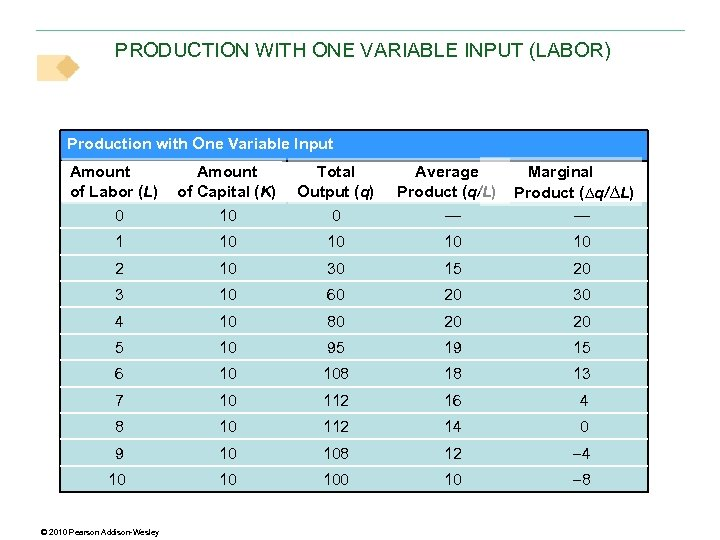PRODUCTION WITH ONE VARIABLE INPUT (LABOR) Production with One Variable Input Amount of Labor (L) Total Output (q) 0 10 0 — 1 10 10 2 10 30 15 20 3 10 60 20 30 4 10 80 20 20 5 10 95 19 15 6 10 108 18 13 7 10 112 16 4 8 10 112 14 0 9 10 108 12 4 10 10 10 8 © 2010 Pearson Addison-Wesley Average Product (q/L) Marginal Product (∆q/∆L) — Amount of Capital (K)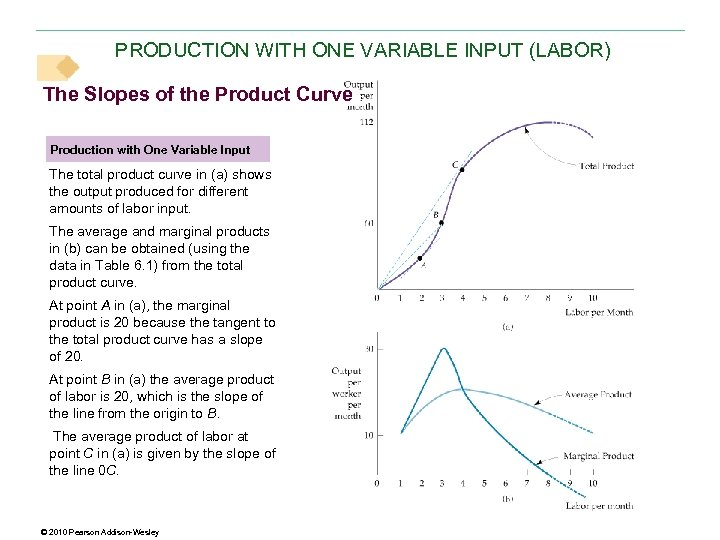PRODUCTION WITH ONE VARIABLE INPUT (LABOR) The Slopes of the Product Curve Production with One Variable Input The total product curve in (a) shows the output produced for different amounts of labor input. The average and marginal products in (b) can be obtained (using the data in Table 6. 1) from the total product curve. At point A in (a), the marginal product is 20 because the tangent to the total product curve has a slope of 20. At point B in (a) the average product of labor is 20, which is the slope of the line from the origin to B. The average product of labor at point C in (a) is given by the slope of the line 0 C. © 2010 Pearson Addison-Wesley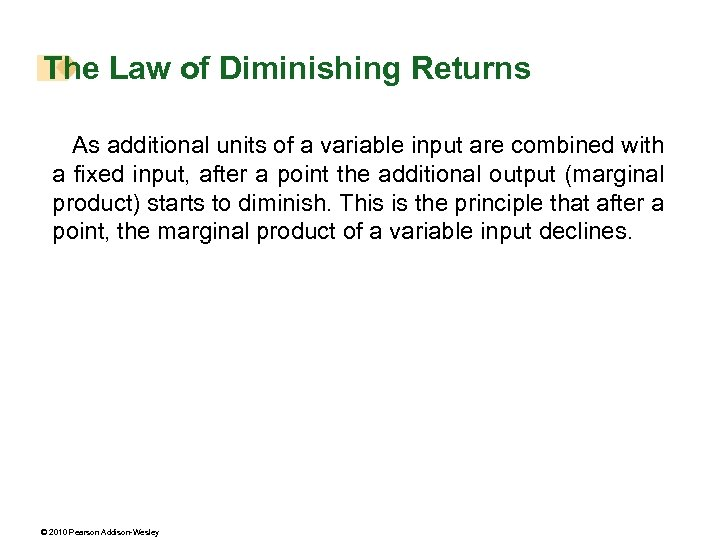The Law of Diminishing Returns As additional units of a variable input are combined with a fixed input, after a point the additional output (marginal product) starts to diminish. This is the principle that after a point, the marginal product of a variable input declines. © 2010 Pearson Addison-Wesley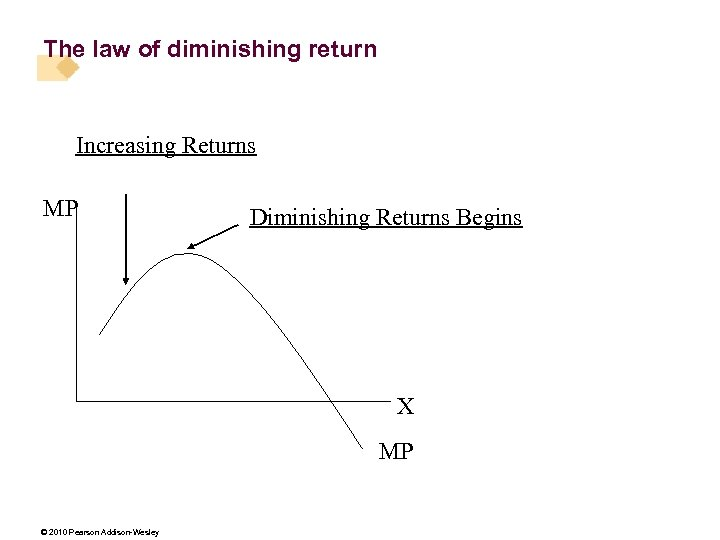The law of diminishing return Increasing Returns MP Diminishing Returns Begins X MP © 2010 Pearson Addison-Wesley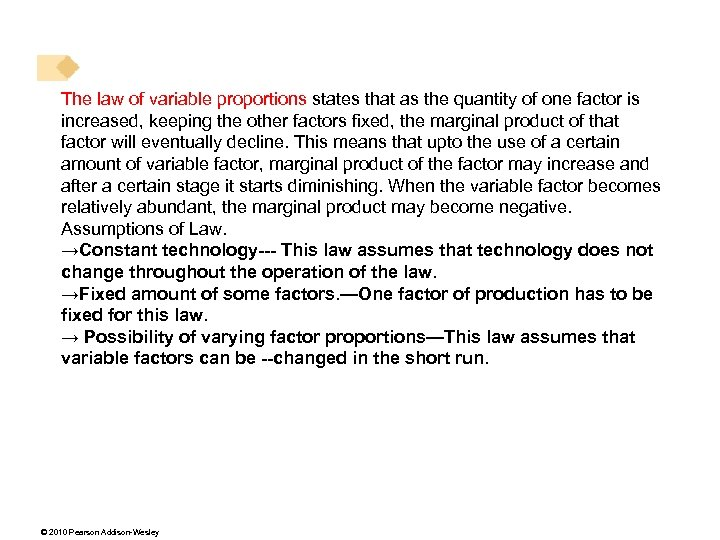The law of variable proportions states that as the quantity of one factor is increased, keeping the other factors fixed, the marginal product of that factor will eventually decline. This means that upto the use of a certain amount of variable factor, marginal product of the factor may increase and after a certain stage it starts diminishing. When the variable factor becomes relatively abundant, the marginal product may become negative. Assumptions of Law. →Constant technology--- This law assumes that technology does not change throughout the operation of the law. →Fixed amount of some factors. —One factor of production has to be fixed for this law. → Possibility of varying factor proportions—This law assumes that variable factors can be --changed in the short run. © 2010 Pearson Addison-Wesley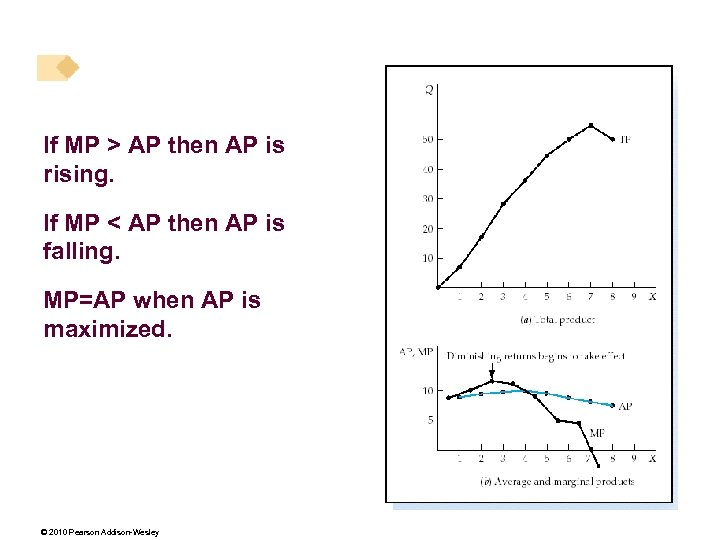If MP > AP then AP is rising. If MP < AP then AP is falling. MP=AP when AP is maximized. © 2010 Pearson Addison-Wesley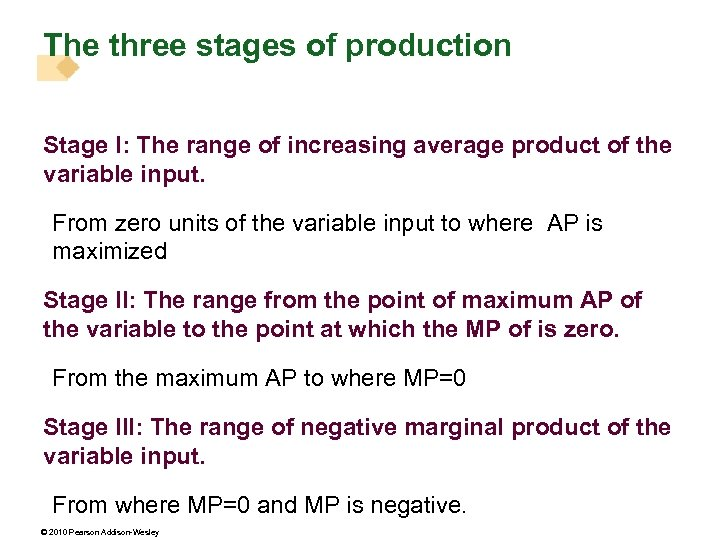The three stages of production Stage I: The range of increasing average product of the variable input. From zero units of the variable input to where AP is maximized Stage II: The range from the point of maximum AP of the variable to the point at which the MP of is zero. From the maximum AP to where MP=0 Stage III: The range of negative marginal product of the variable input. From where MP=0 and MP is negative. © 2010 Pearson Addison-Wesley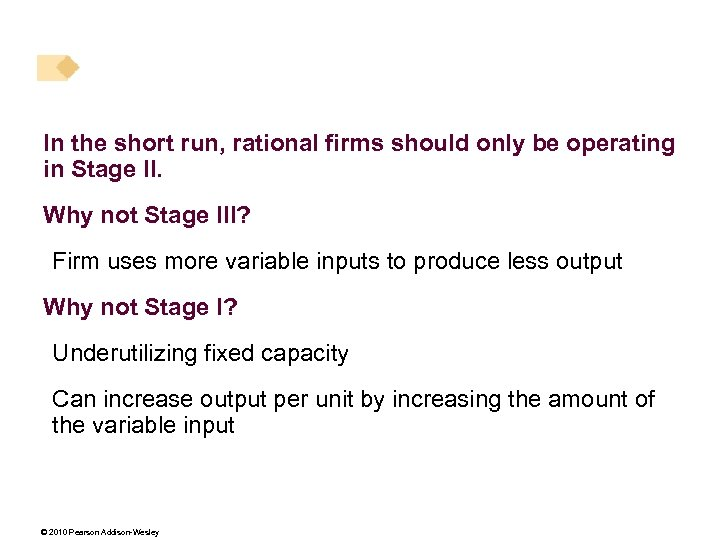In the short run, rational firms should only be operating in Stage II. Why not Stage III? Firm uses more variable inputs to produce less output Why not Stage I? Underutilizing fixed capacity Can increase output per unit by increasing the amount of the variable input © 2010 Pearson Addison-Wesley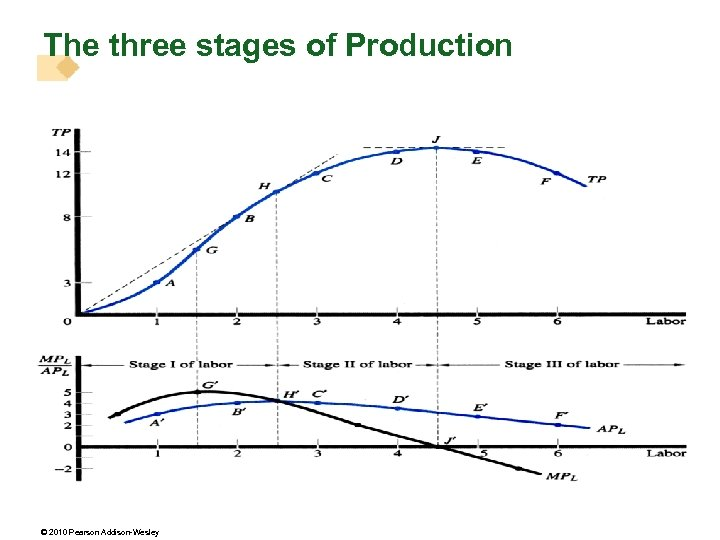The three stages of Production © 2010 Pearson Addison-Wesley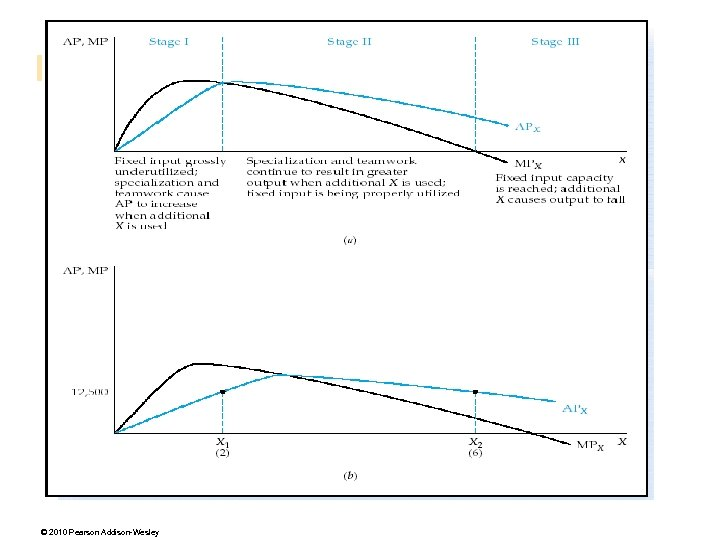© 2010 Pearson Addison-Wesley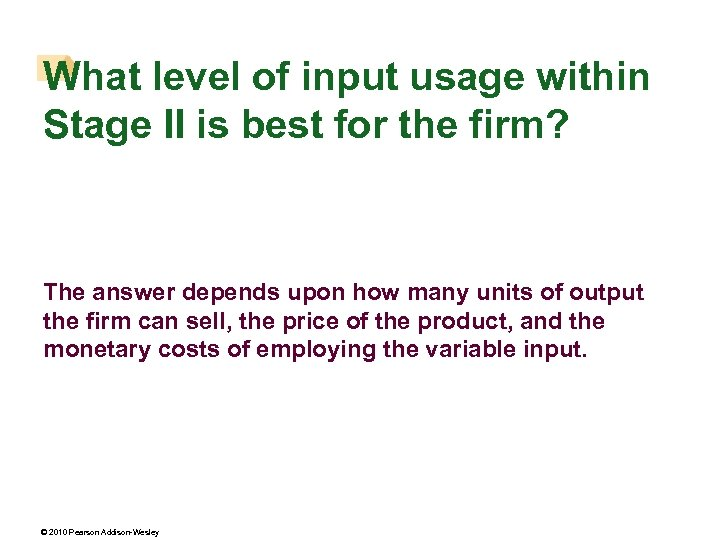What level of input usage within Stage II is best for the firm? The answer depends upon how many units of output the firm can sell, the price of the product, and the monetary costs of employing the variable input. © 2010 Pearson Addison-Wesley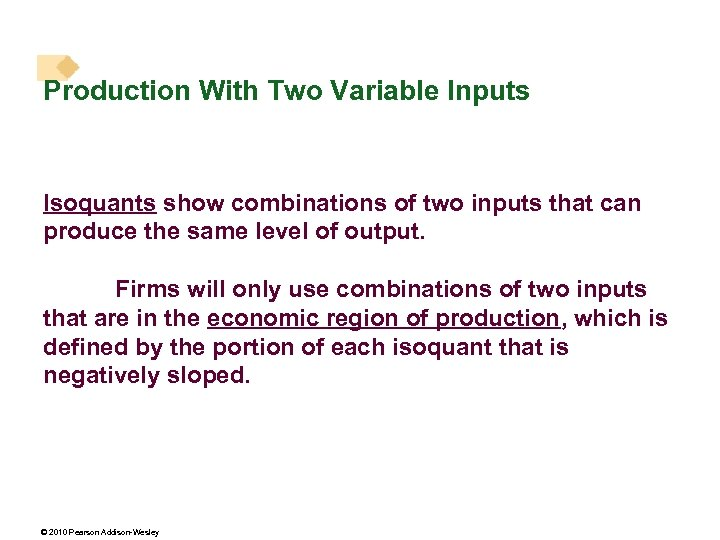Production With Two Variable Inputs Isoquants show combinations of two inputs that can produce the same level of output. Firms will only use combinations of two inputs that are in the economic region of production, which is defined by the portion of each isoquant that is negatively sloped. © 2010 Pearson Addison-Wesley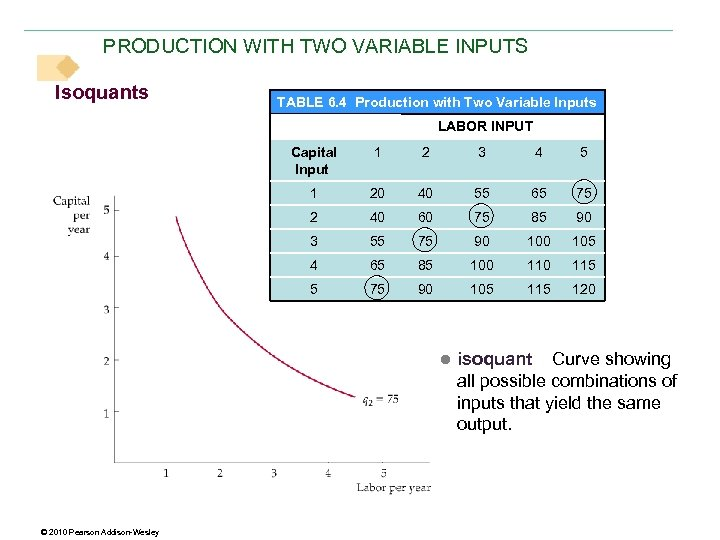PRODUCTION WITH TWO VARIABLE INPUTS Isoquants TABLE 6. 4 Production with Two Variable Inputs LABOR INPUT Capital Input 1 2 3 4 5 1 20 40 55 65 75 2 40 60 75 85 90 3 55 75 90 105 4 65 85 100 115 5 75 90 105 115 120 ● isoquant Curve showing all possible combinations of inputs that yield the same output. © 2010 Pearson Addison-Wesley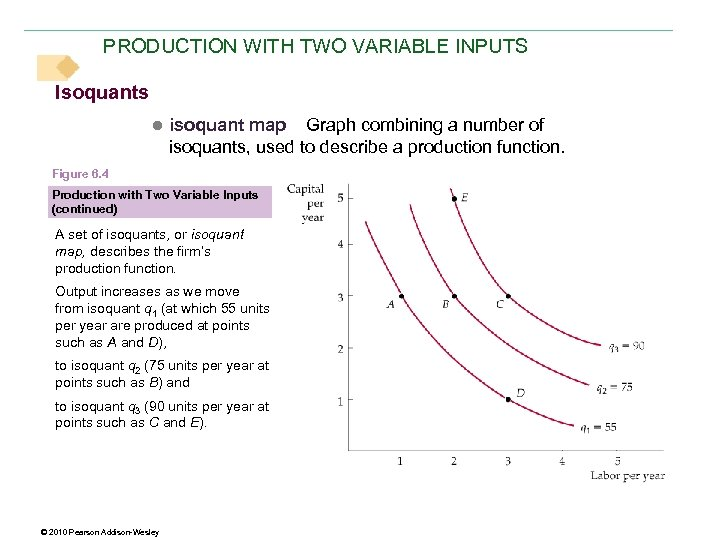PRODUCTION WITH TWO VARIABLE INPUTS Isoquants ● isoquant map Graph combining a number of isoquants, used to describe a production function. Figure 6. 4 Production with Two Variable Inputs (continued) A set of isoquants, or isoquant map, describes the firm’s production function. Output increases as we move from isoquant q 1 (at which 55 units per year are produced at points such as A and D), to isoquant q 2 (75 units per year at points such as B) and to isoquant q 3 (90 units per year at points such as C and E). © 2010 Pearson Addison-Wesley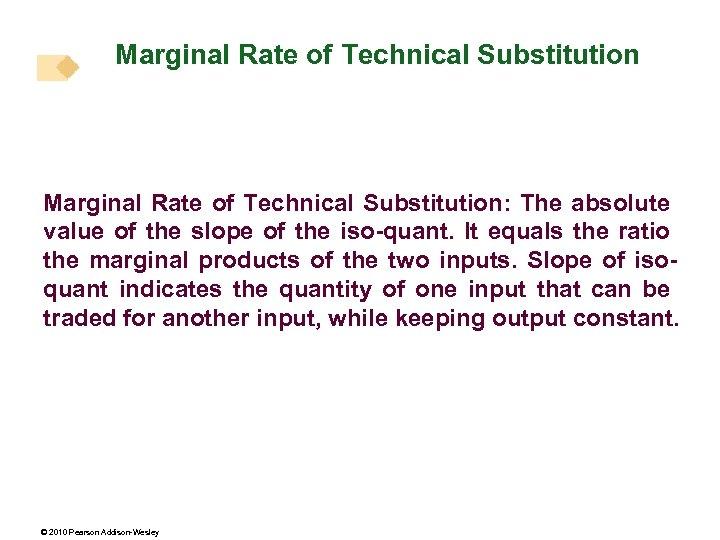Marginal Rate of Technical Substitution: The absolute value of the slope of the iso-quant. It equals the ratio the marginal products of the two inputs. Slope of isoquant indicates the quantity of one input that can be traded for another input, while keeping output constant. © 2010 Pearson Addison-Wesley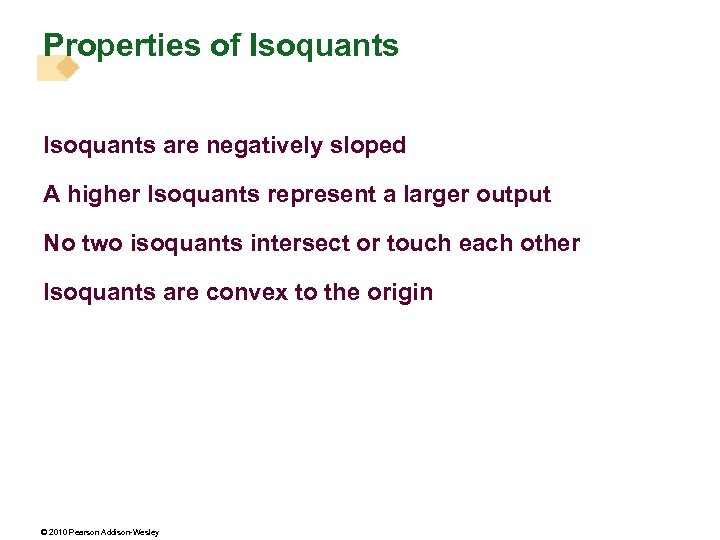Properties of Isoquants are negatively sloped A higher Isoquants represent a larger output No two isoquants intersect or touch each other Isoquants are convex to the origin © 2010 Pearson Addison-Wesley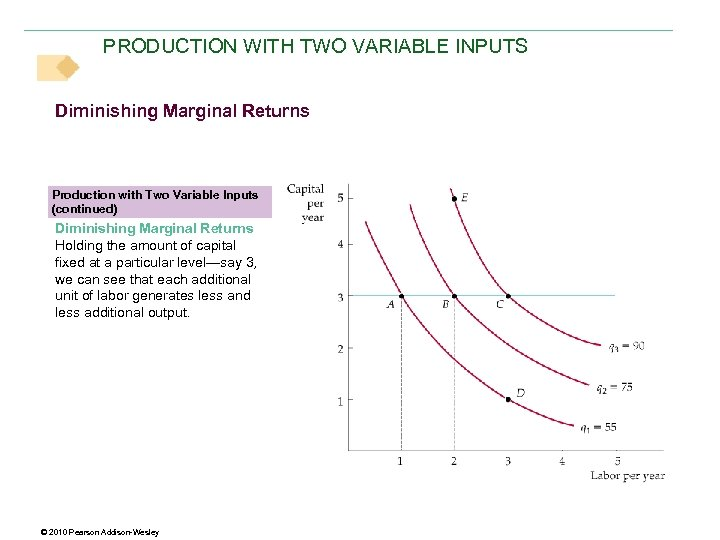PRODUCTION WITH TWO VARIABLE INPUTS Diminishing Marginal Returns Production with Two Variable Inputs (continued) Diminishing Marginal Returns Holding the amount of capital fixed at a particular level—say 3, we can see that each additional unit of labor generates less and less additional output. © 2010 Pearson Addison-Wesley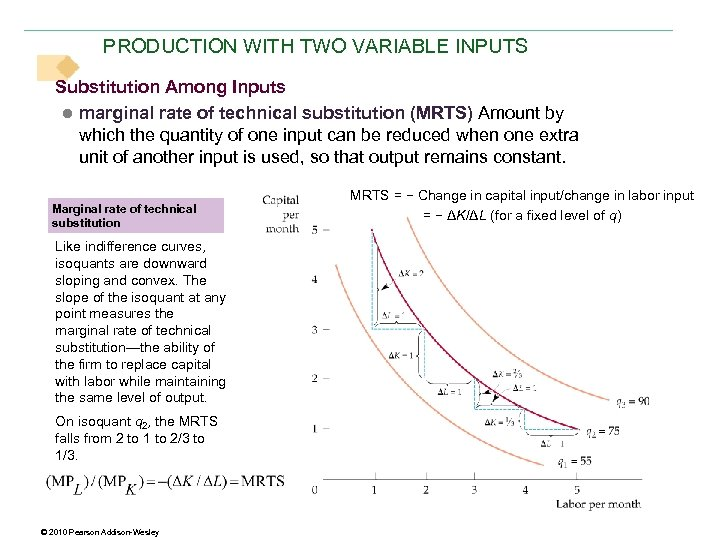PRODUCTION WITH TWO VARIABLE INPUTS Substitution Among Inputs ● marginal rate of technical substitution (MRTS) Amount by which the quantity of one input can be reduced when one extra unit of another input is used, so that output remains constant. Marginal rate of technical substitution Like indifference curves, isoquants are downward sloping and convex. The slope of the isoquant at any point measures the marginal rate of technical substitution—the ability of the firm to replace capital with labor while maintaining the same level of output. On isoquant q 2, the MRTS falls from 2 to 1 to 2/3 to 1/3. © 2010 Pearson Addison-Wesley MRTS = − Change in capital input/change in labor input = − ΔK/ΔL (for a fixed level of q)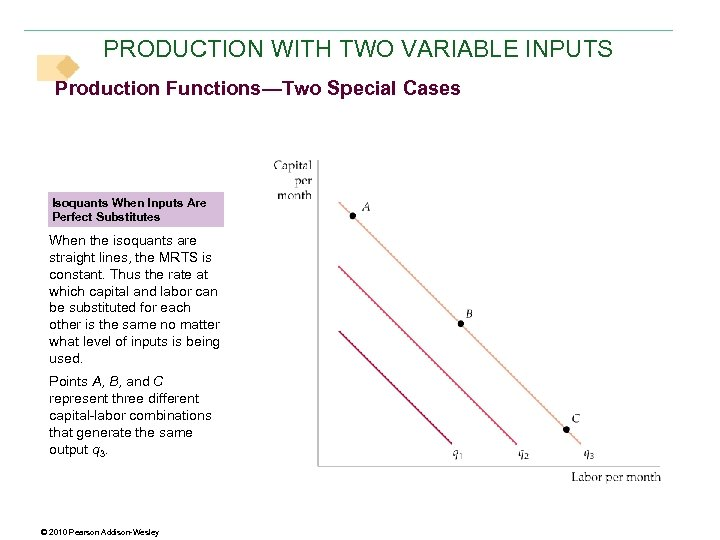PRODUCTION WITH TWO VARIABLE INPUTS Production Functions—Two Special Cases Isoquants When Inputs Are Perfect Substitutes When the isoquants are straight lines, the MRTS is constant. Thus the rate at which capital and labor can be substituted for each other is the same no matter what level of inputs is being used. Points A, B, and C represent three different capital-labor combinations that generate the same output q 3. © 2010 Pearson Addison-Wesley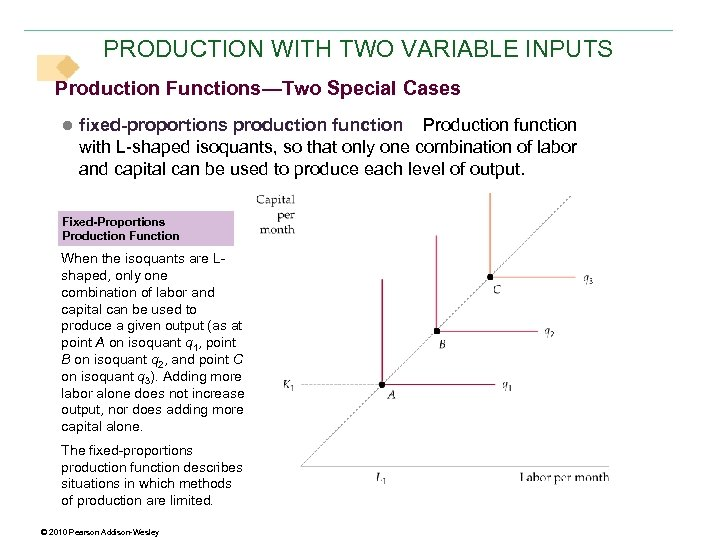PRODUCTION WITH TWO VARIABLE INPUTS Production Functions—Two Special Cases ● fixed-proportions production function Production function with L-shaped isoquants, so that only one combination of labor and capital can be used to produce each level of output. Fixed-Proportions Production Function When the isoquants are Lshaped, only one combination of labor and capital can be used to produce a given output (as at point A on isoquant q 1, point B on isoquant q 2, and point C on isoquant q 3). Adding more labor alone does not increase output, nor does adding more capital alone. The fixed-proportions production function describes situations in which methods of production are limited. © 2010 Pearson Addison-Wesley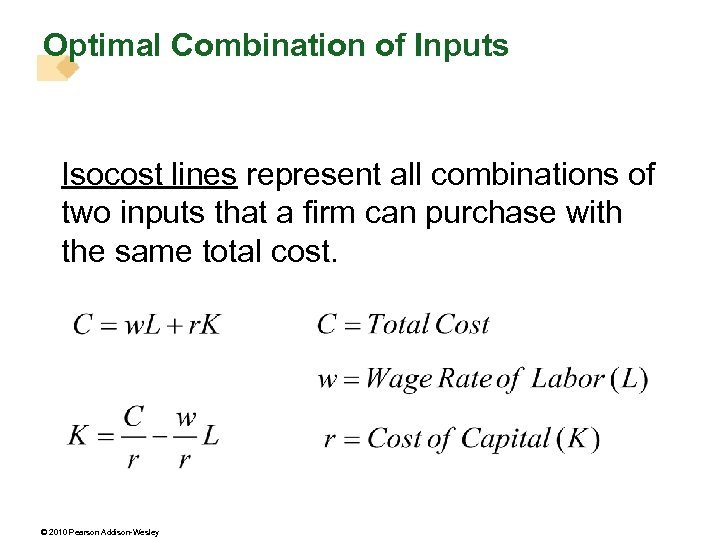Optimal Combination of Inputs Isocost lines represent all combinations of two inputs that a firm can purchase with the same total cost. © 2010 Pearson Addison-Wesley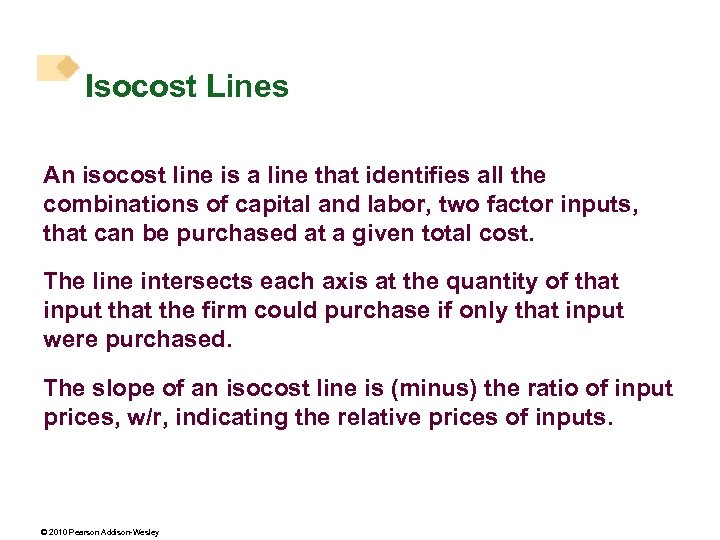Isocost Lines An isocost line is a line that identifies all the combinations of capital and labor, two factor inputs, that can be purchased at a given total cost. The line intersects each axis at the quantity of that input that the firm could purchase if only that input were purchased. The slope of an isocost line is (minus) the ratio of input prices, w/r, indicating the relative prices of inputs. © 2010 Pearson Addison-Wesley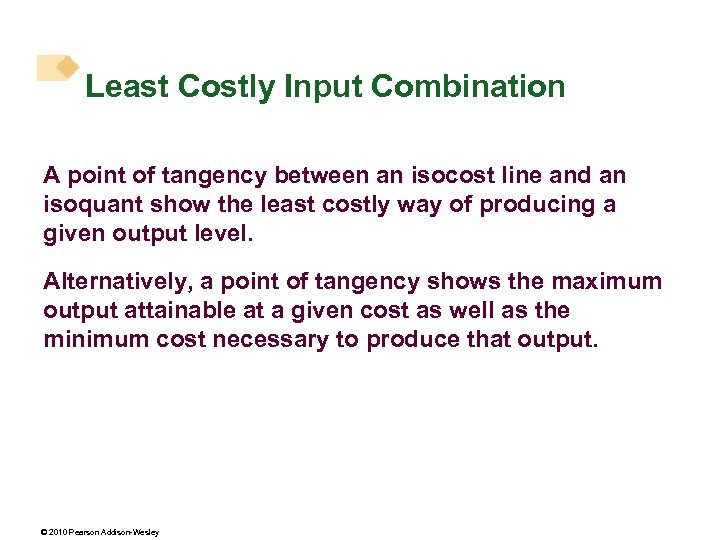Least Costly Input Combination A point of tangency between an isocost line and an isoquant show the least costly way of producing a given output level. Alternatively, a point of tangency shows the maximum output attainable at a given cost as well as the minimum cost necessary to produce that output. © 2010 Pearson Addison-Wesley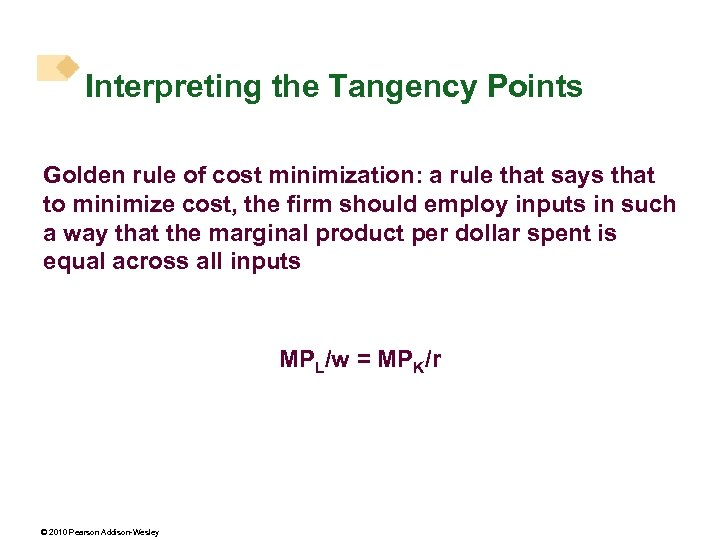Interpreting the Tangency Points Golden rule of cost minimization: a rule that says that to minimize cost, the firm should employ inputs in such a way that the marginal product per dollar spent is equal across all inputs MPL/w = MPK/r © 2010 Pearson Addison-Wesley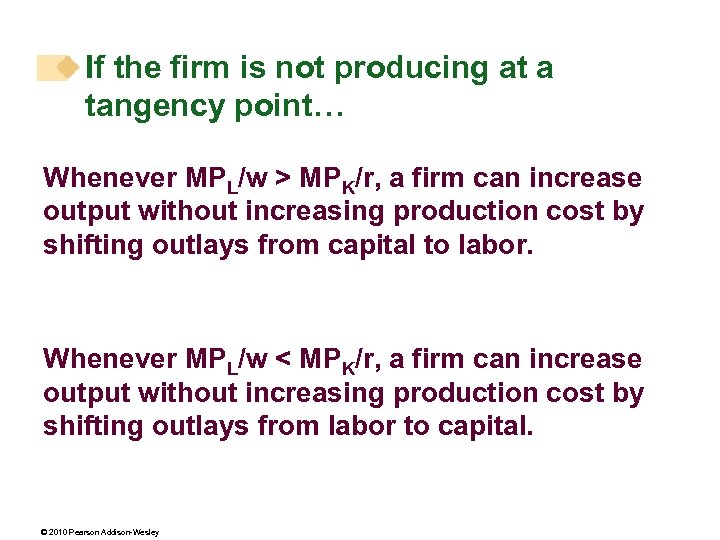If the firm is not producing at a tangency point… Whenever MPL/w > MPK/r, a firm can increase output without increasing production cost by shifting outlays from capital to labor. Whenever MPL/w < MPK/r, a firm can increase output without increasing production cost by shifting outlays from labor to capital. © 2010 Pearson Addison-Wesley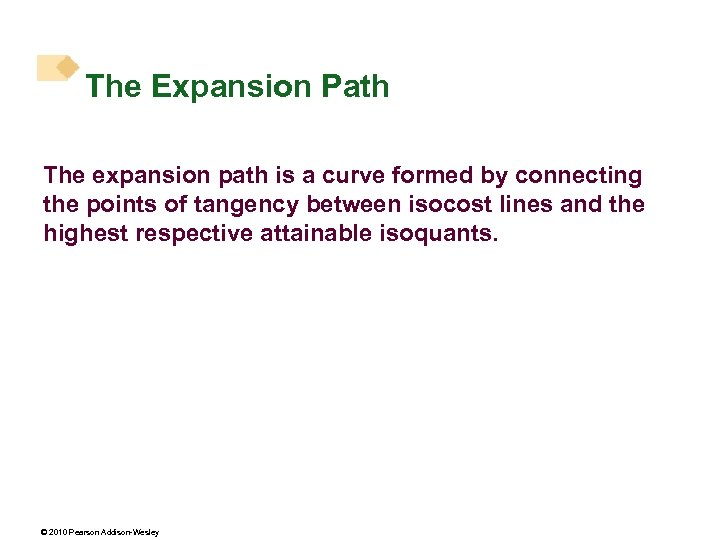The Expansion Path The expansion path is a curve formed by connecting the points of tangency between isocost lines and the highest respective attainable isoquants. © 2010 Pearson Addison-Wesley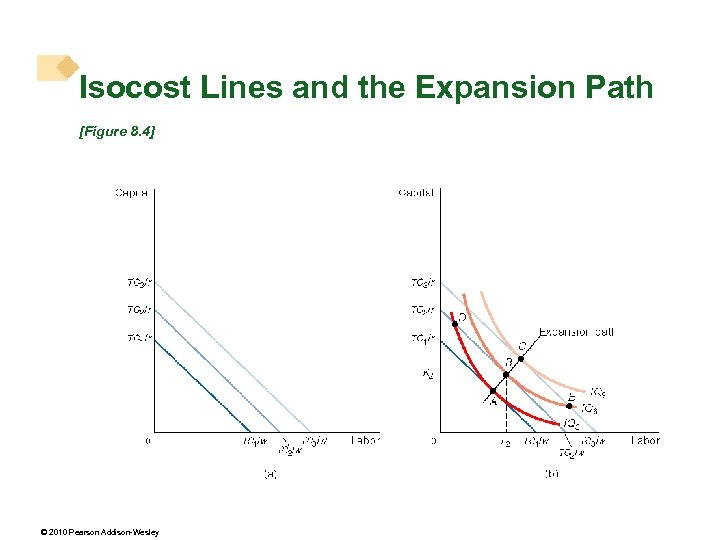Isocost Lines and the Expansion Path [Figure 8. 4] © 2010 Pearson Addison-Wesley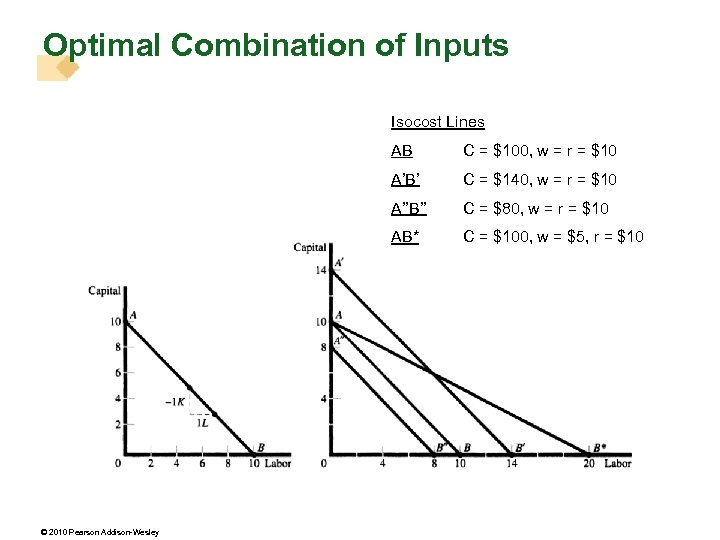Optimal Combination of Inputs Isocost Lines AB A’B’ C = \$140, w = r = \$10 A’’B’’ C = \$80, w = r = \$10 AB* © 2010 Pearson Addison-Wesley C = \$100, w = r = \$10 C = \$100, w = \$5, r = \$10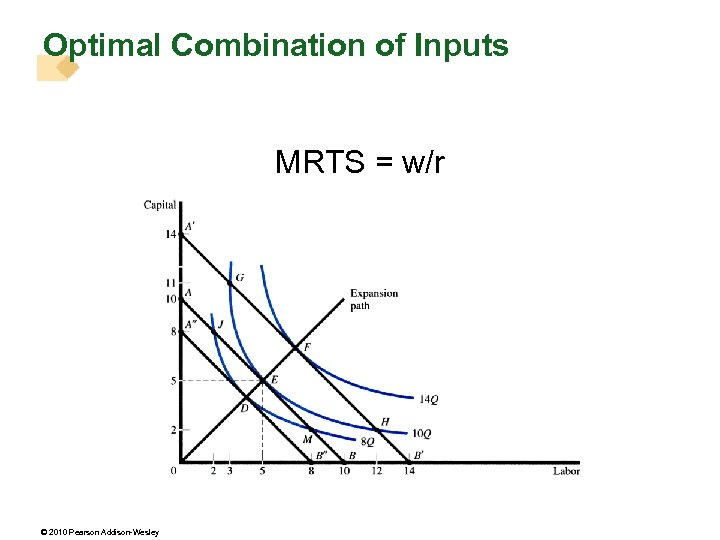Optimal Combination of Inputs MRTS = w/r © 2010 Pearson Addison-Wesley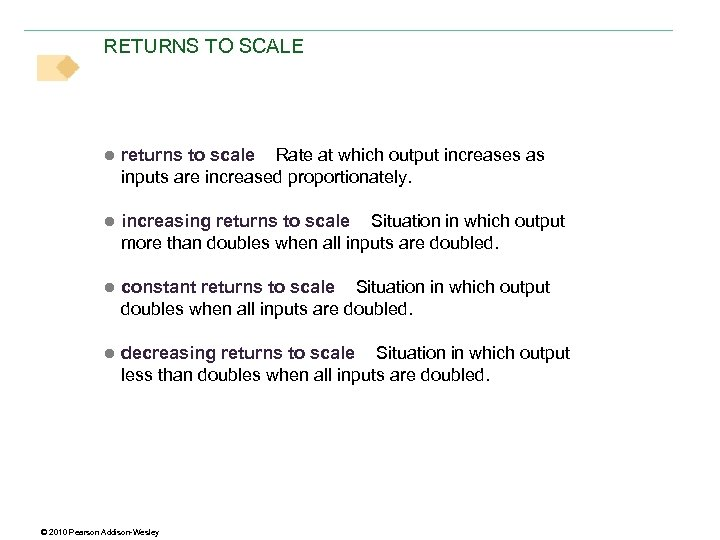RETURNS TO SCALE ● returns to scale Rate at which output increases as inputs are increased proportionately. ● increasing returns to scale Situation in which output more than doubles when all inputs are doubled. ● constant returns to scale Situation in which output doubles when all inputs are doubled. ● decreasing returns to scale Situation in which output less than doubles when all inputs are doubled. © 2010 Pearson Addison-Wesley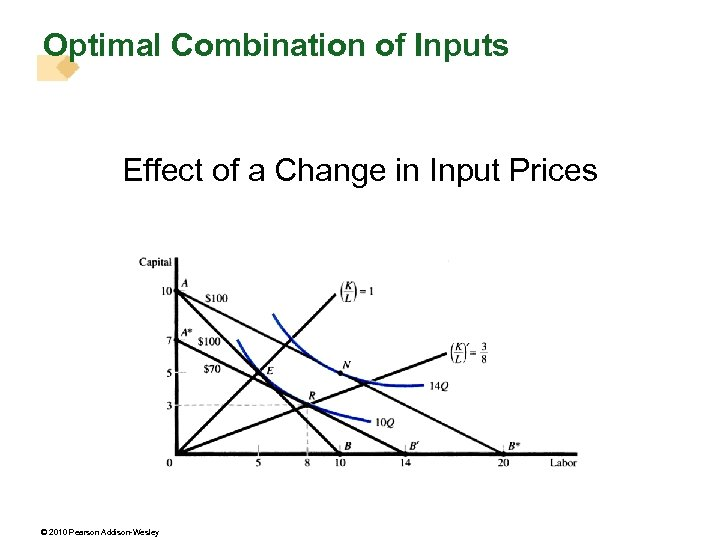Optimal Combination of Inputs Effect of a Change in Input Prices © 2010 Pearson Addison-Wesley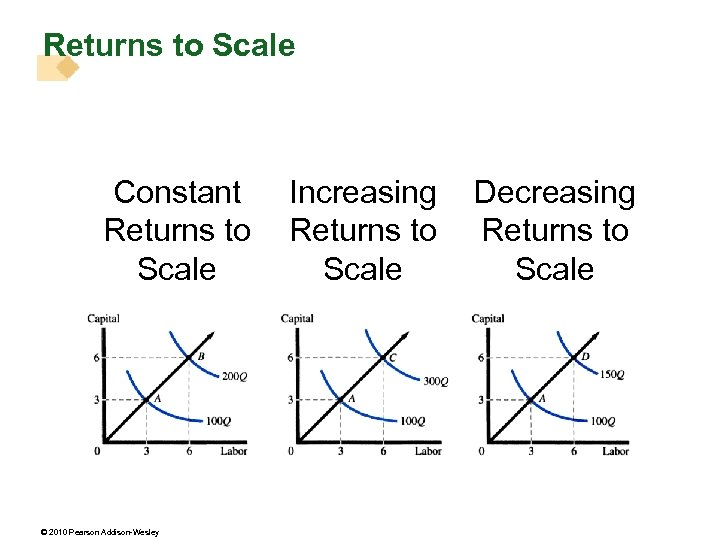Returns to Scale Constant Returns to Scale © 2010 Pearson Addison-Wesley Increasing Returns to Scale Decreasing Returns to Scale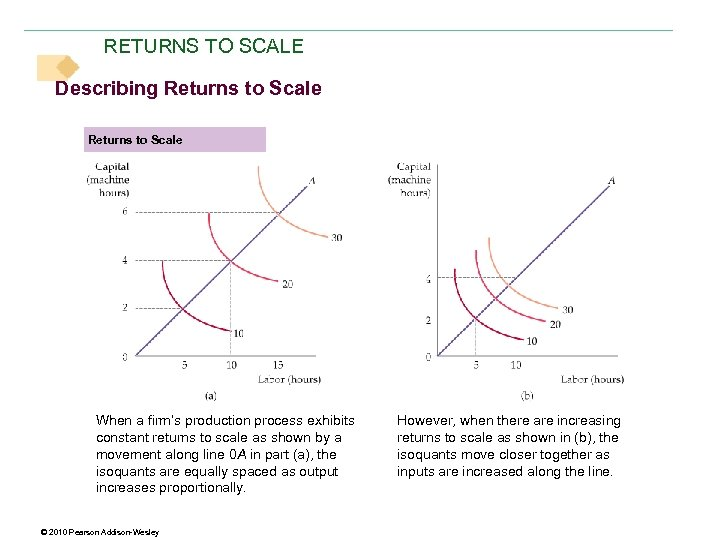RETURNS TO SCALE Describing Returns to Scale When a firm’s production process exhibits constant returns to scale as shown by a movement along line 0 A in part (a), the isoquants are equally spaced as output increases proportionally. © 2010 Pearson Addison-Wesley However, when there are increasing returns to scale as shown in (b), the isoquants move closer together as inputs are increased along the line.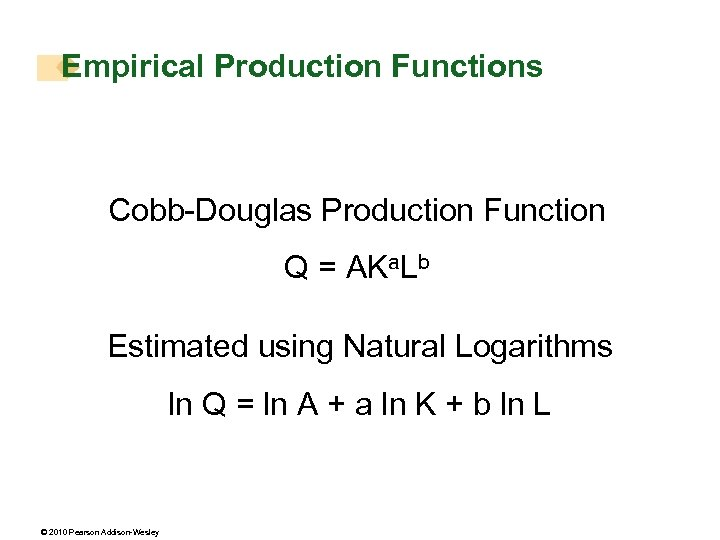Empirical Production Functions Cobb-Douglas Production Function Q = AKa. Lb Estimated using Natural Logarithms ln Q = ln A + a ln K + b ln L © 2010 Pearson Addison-Wesley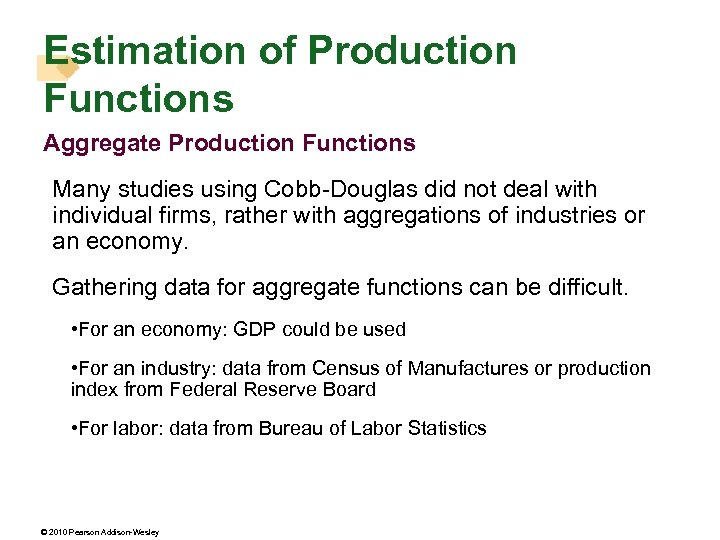Estimation of Production Functions Aggregate Production Functions Many studies using Cobb-Douglas did not deal with individual firms, rather with aggregations of industries or an economy. Gathering data for aggregate functions can be difficult. • For an economy: GDP could be used • For an industry: data from Census of Manufactures or production index from Federal Reserve Board • For labor: data from Bureau of Labor Statistics © 2010 Pearson Addison-Wesley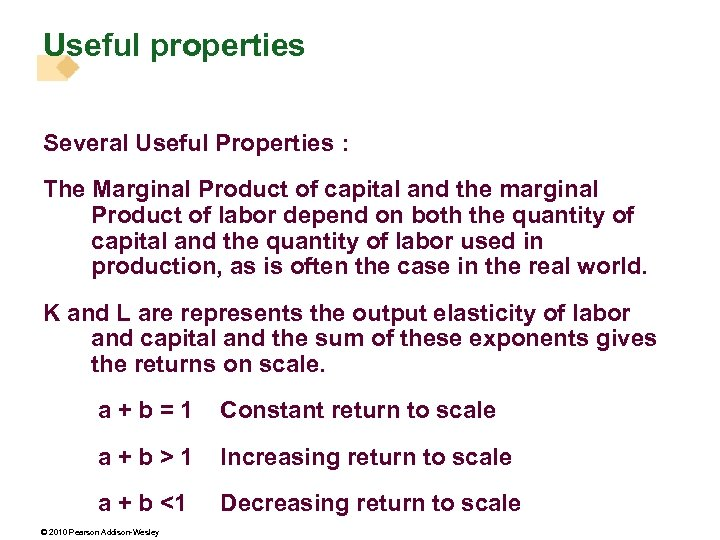Useful properties Several Useful Properties : The Marginal Product of capital and the marginal Product of labor depend on both the quantity of capital and the quantity of labor used in production, as is often the case in the real world. K and L are represents the output elasticity of labor and capital and the sum of these exponents gives the returns on scale. a+b=1 Constant return to scale a+b>1 Increasing return to scale a + b <1 Decreasing return to scale © 2010 Pearson Addison-Wesley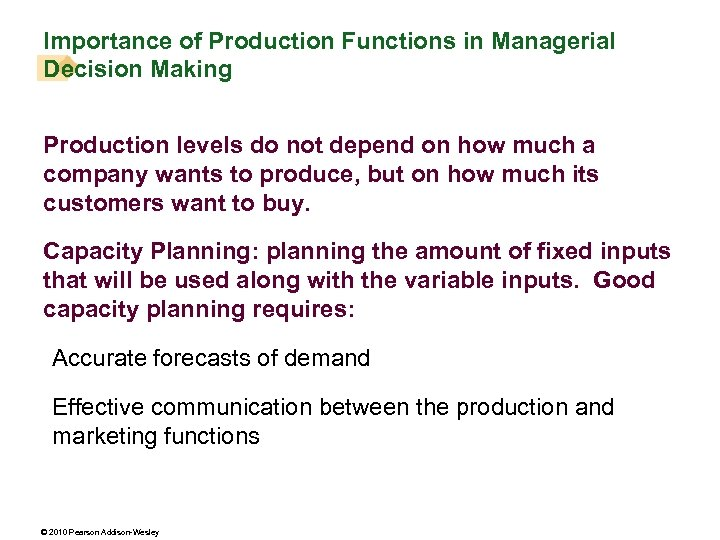Importance of Production Functions in Managerial Decision Making Production levels do not depend on how much a company wants to produce, but on how much its customers want to buy. Capacity Planning: planning the amount of fixed inputs that will be used along with the variable inputs. Good capacity planning requires: Accurate forecasts of demand Effective communication between the production and marketing functions © 2010 Pearson Addison-Wesley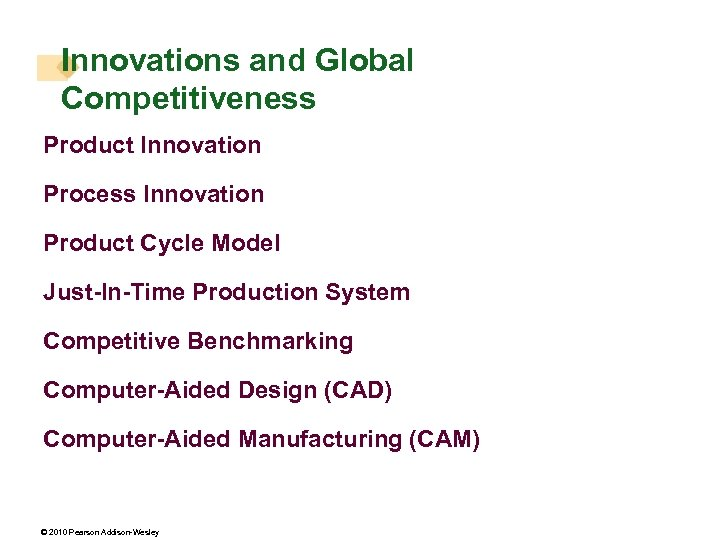Innovations and Global Competitiveness Product Innovation Process Innovation Product Cycle Model Just-In-Time Production System Competitive Benchmarking Computer-Aided Design (CAD) Computer-Aided Manufacturing (CAM) © 2010 Pearson Addison-Wesley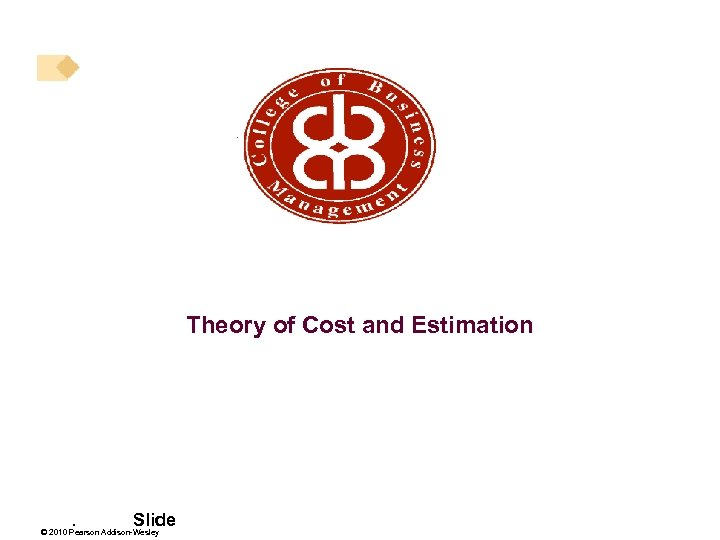Theory of Cost and Estimation . Slide © 2010 Pearson Addison-WesleyThe Nature of Costs Explicit Costs Accounting Costs arise from transactions in which the firm purchases inputs or the services of inputs from other parties Economic Costs Implicit Costs Explicit cost Alternative or Opportunity Costs. Slide 56 © 2010 Pearson Addison-Wesley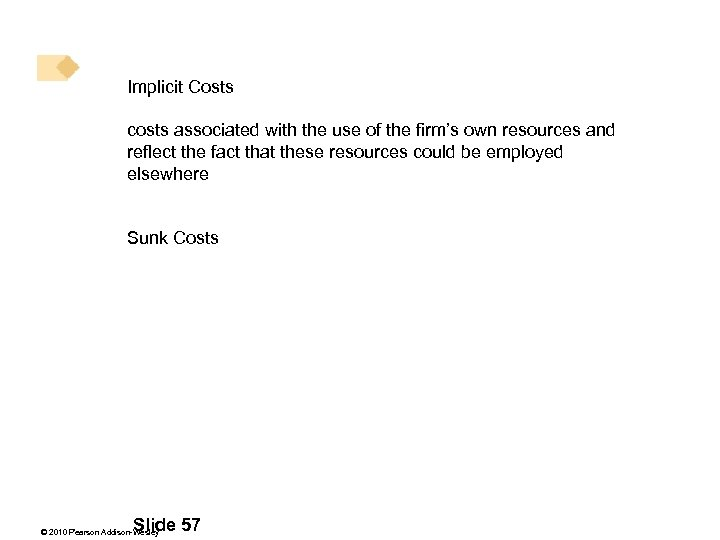Implicit Costs costs associated with the use of the firm’s own resources and reflect the fact that these resources could be employed elsewhere Sunk Costs . Slide 57 © 2010 Pearson Addison-Wesley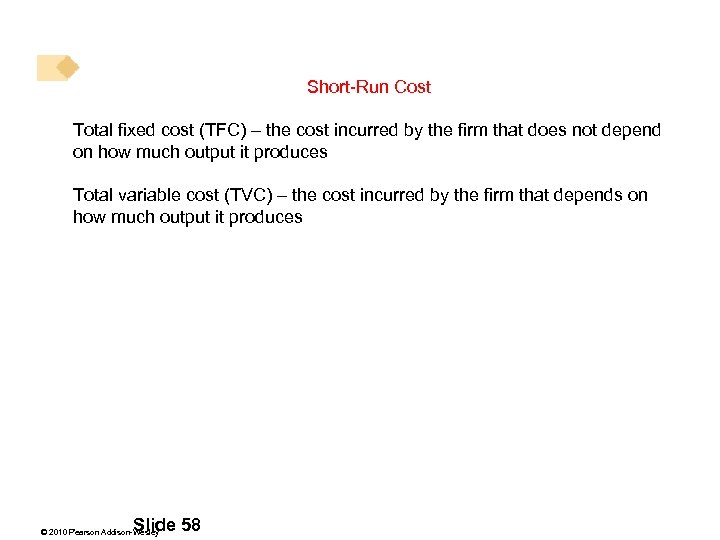Short-Run Cost Total fixed cost (TFC) – the cost incurred by the firm that does not depend on how much output it produces Total variable cost (TVC) – the cost incurred by the firm that depends on how much output it produces . Slide 58 © 2010 Pearson Addison-Wesley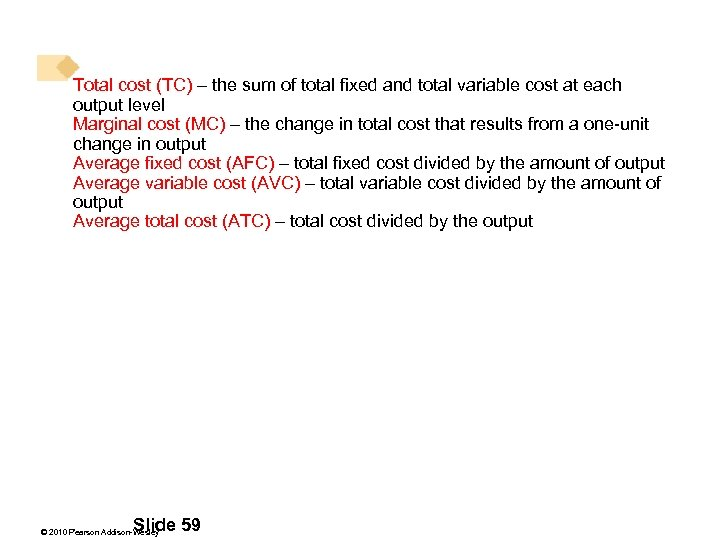Total cost (TC) – the sum of total fixed and total variable cost at each output level Marginal cost (MC) – the change in total cost that results from a one-unit change in output Average fixed cost (AFC) – total fixed cost divided by the amount of output Average variable cost (AVC) – total variable cost divided by the amount of output Average total cost (ATC) – total cost divided by the output . Slide 59 © 2010 Pearson Addison-Wesley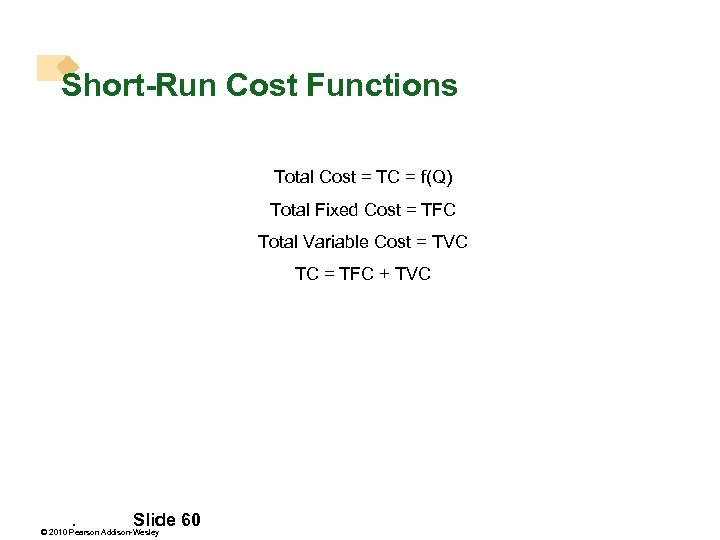Short-Run Cost Functions Total Cost = TC = f(Q) Total Fixed Cost = TFC Total Variable Cost = TVC TC = TFC + TVC . Slide 60 © 2010 Pearson Addison-Wesley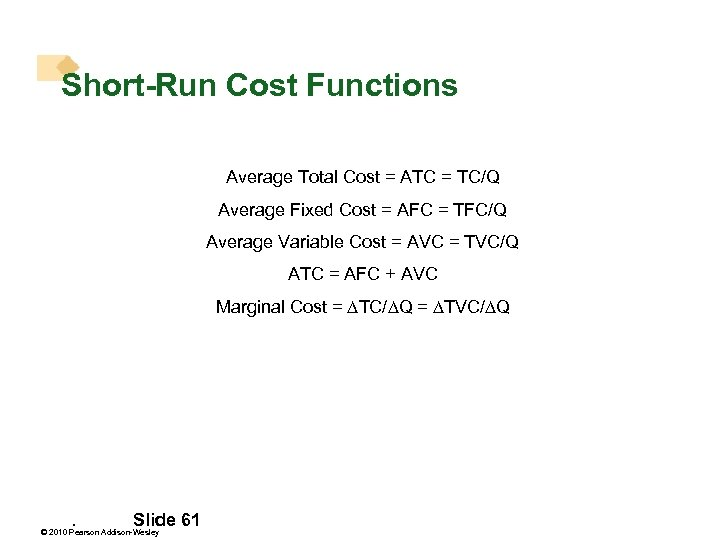Short-Run Cost Functions Average Total Cost = ATC = TC/Q Average Fixed Cost = AFC = TFC/Q Average Variable Cost = AVC = TVC/Q ATC = AFC + AVC Marginal Cost = TC/ Q = TVC/ Q . Slide 61 © 2010 Pearson Addison-Wesley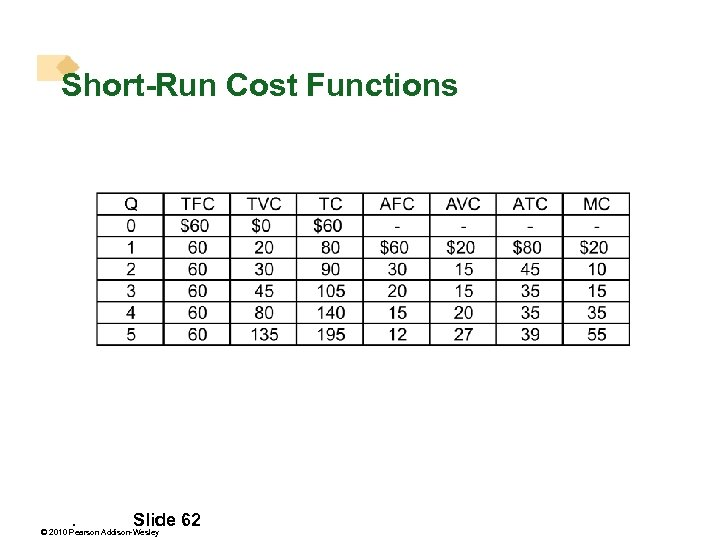Short-Run Cost Functions . Slide 62 © 2010 Pearson Addison-Wesley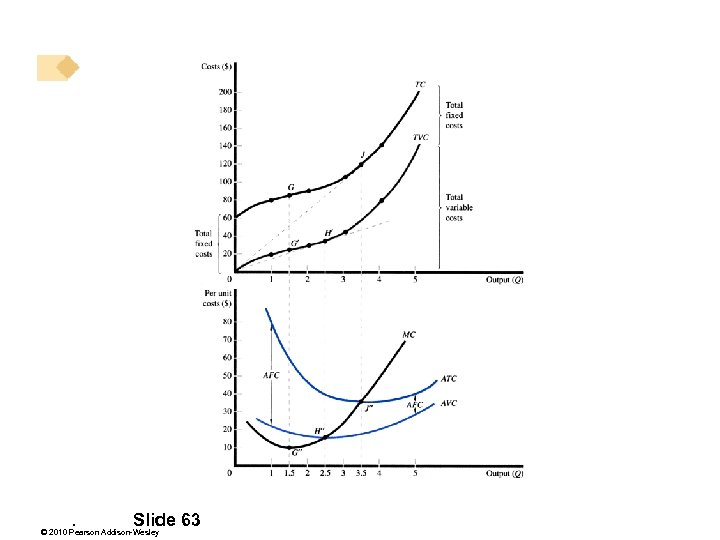. Slide 63 © 2010 Pearson Addison-Wesley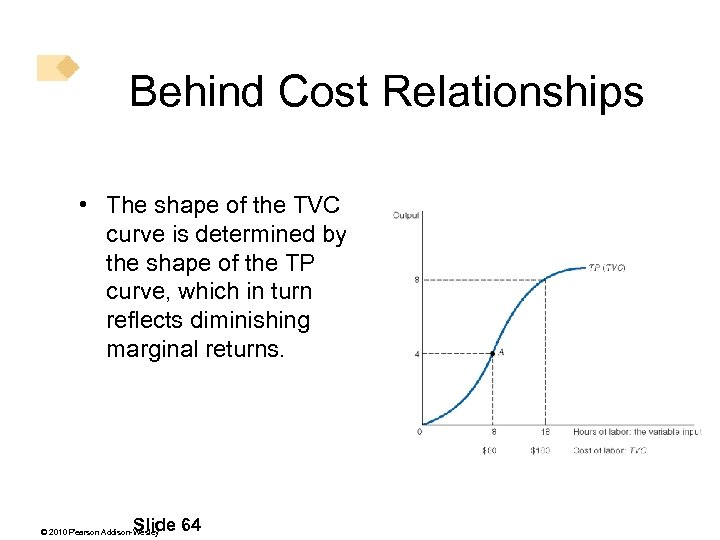Behind Cost Relationships • The shape of the TVC curve is determined by the shape of the TP curve, which in turn reflects diminishing marginal returns. . Slide 64 © 2010 Pearson Addison-Wesley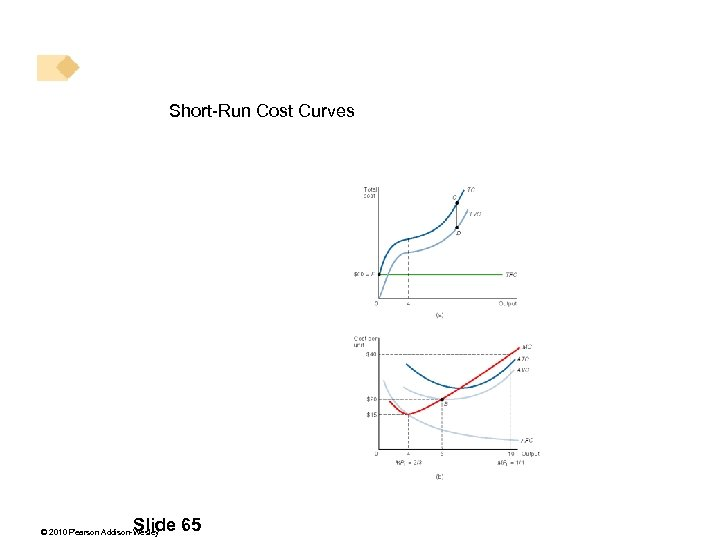Short-Run Cost Curves . Slide 65 © 2010 Pearson Addison-Wesley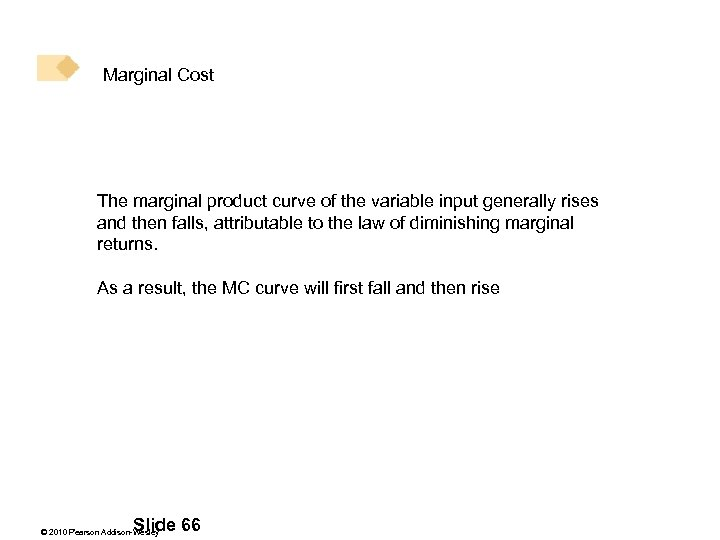Marginal Cost The marginal product curve of the variable input generally rises and then falls, attributable to the law of diminishing marginal returns. As a result, the MC curve will first fall and then rise . Slide 66 © 2010 Pearson Addison-WesleyAverage Cost The average product curve rises, reaches a maximum, and then falls, due to the law of diminishing marginal productivity. As a result, the AVC curve will fall and then rise. The AFC curve declines over the entire range of output as the amount of total fixed cost is spread over ever-larger rates of output. The ATC curve is the sum of AFC and AVC. It measures the average unit cost of all inputs, both fixed and variable, and must also be U-shaped. . Slide 67 © 2010 Pearson Addison-Wesley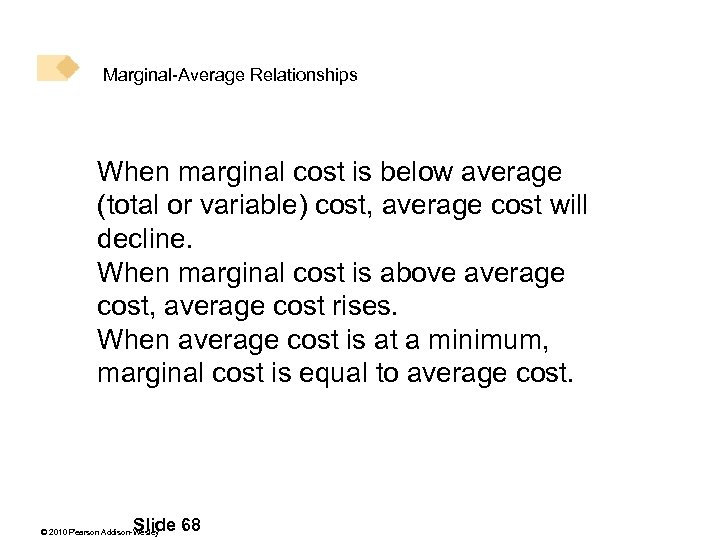Marginal-Average Relationships When marginal cost is below average (total or variable) cost, average cost will decline. When marginal cost is above average cost, average cost rises. When average cost is at a minimum, marginal cost is equal to average cost. . Slide 68 © 2010 Pearson Addison-Wesley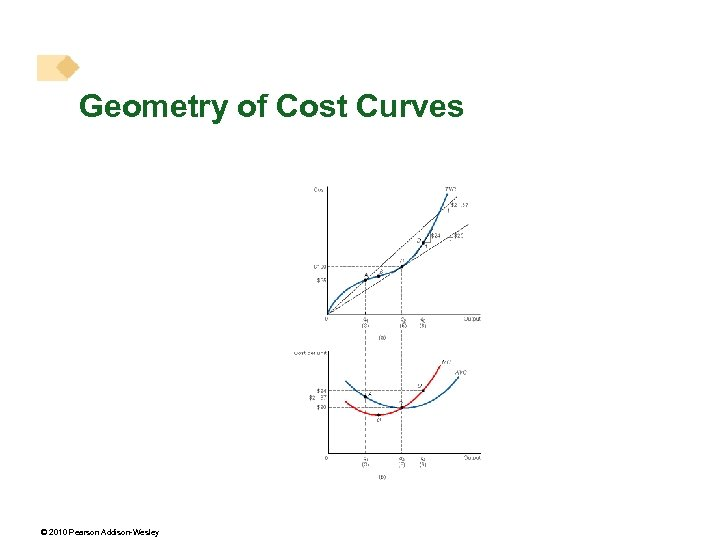Geometry of Cost Curves © 2010 Pearson Addison-Wesley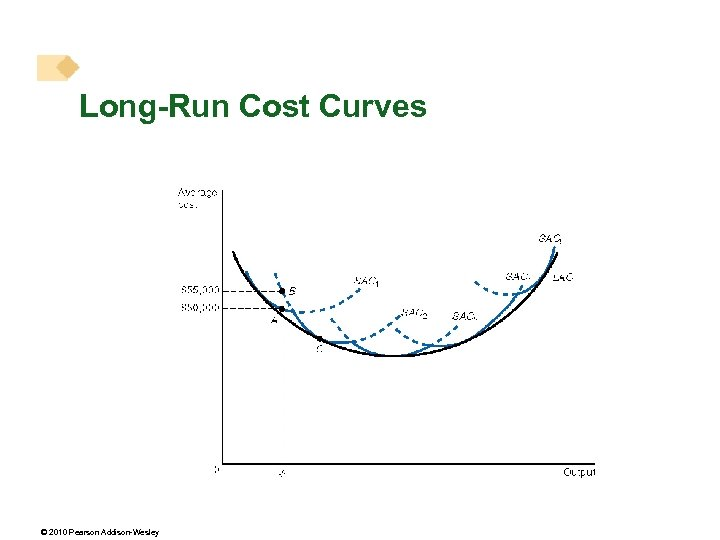Long-Run Cost Curves © 2010 Pearson Addison-Wesley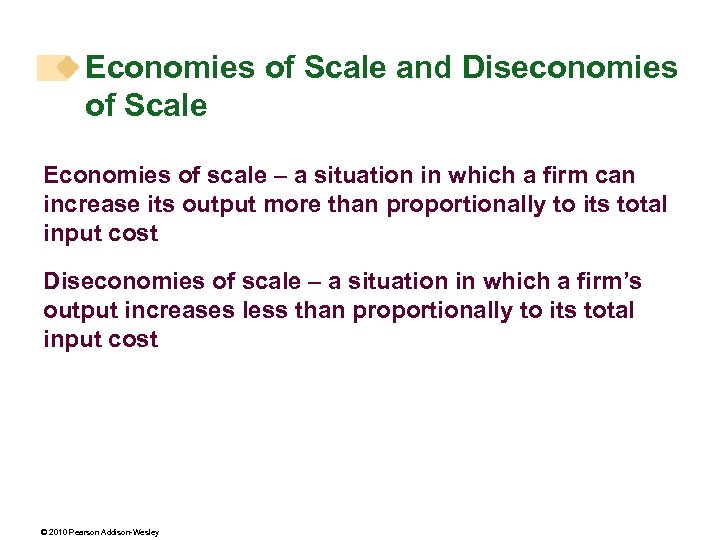Economies of Scale and Diseconomies of Scale Economies of scale – a situation in which a firm can increase its output more than proportionally to its total input cost Diseconomies of scale – a situation in which a firm’s output increases less than proportionally to its total input cost © 2010 Pearson Addison-Wesley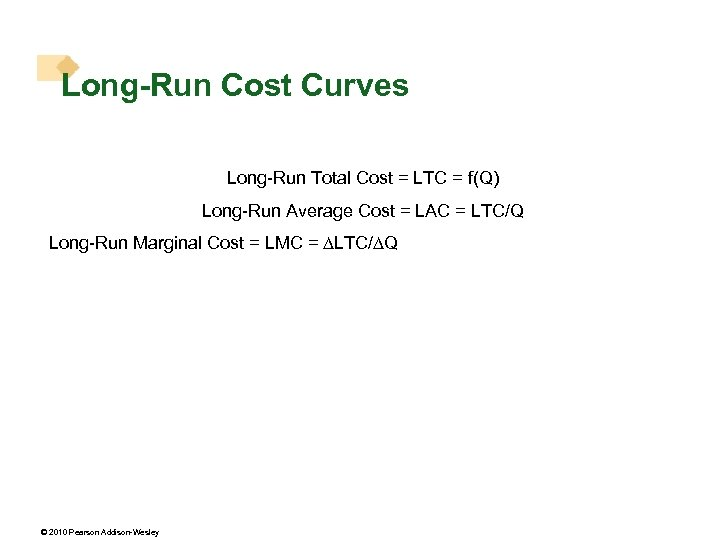Long-Run Cost Curves Long-Run Total Cost = LTC = f(Q) Long-Run Average Cost = LAC = LTC/Q Long-Run Marginal Cost = LMC = LTC/ Q © 2010 Pearson Addison-Wesley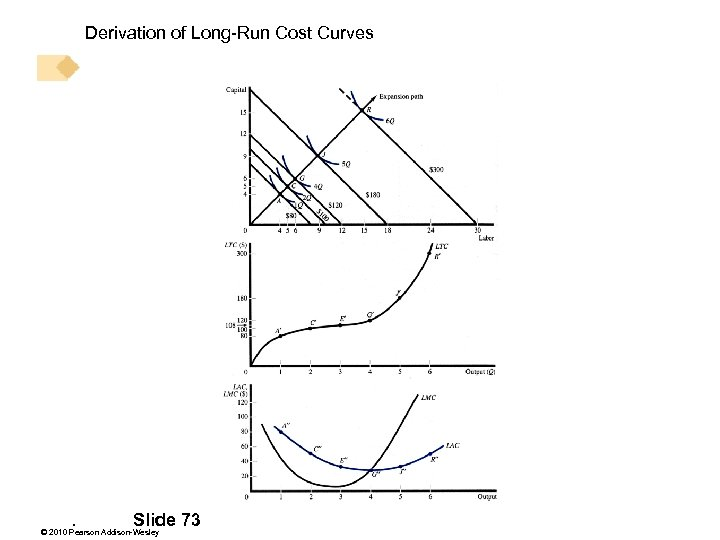Derivation of Long-Run Cost Curves . Slide 73 © 2010 Pearson Addison-Wesley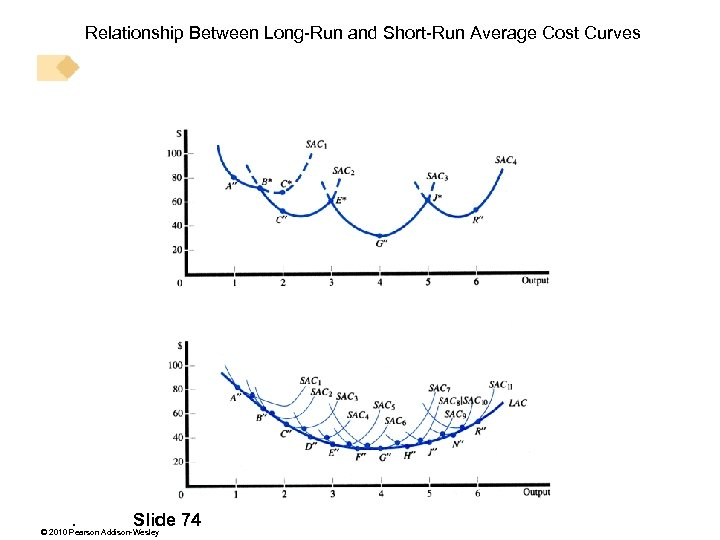Relationship Between Long-Run and Short-Run Average Cost Curves . Slide 74 © 2010 Pearson Addison-Wesley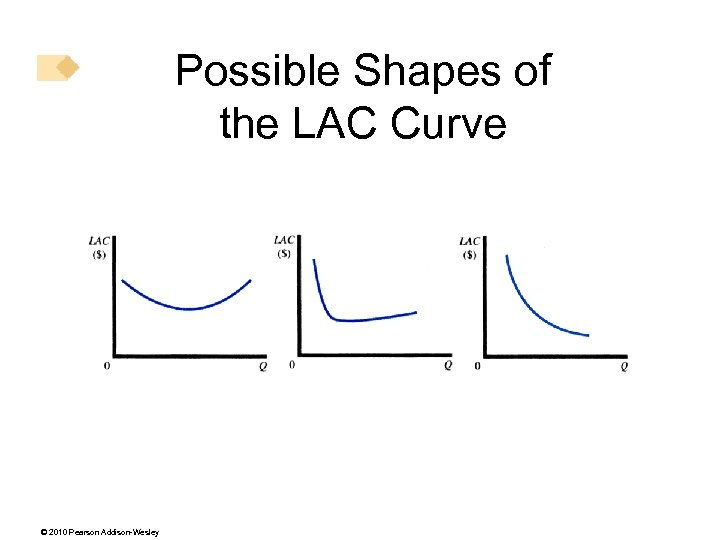Possible Shapes of the LAC Curve © 2010 Pearson Addison-Wesley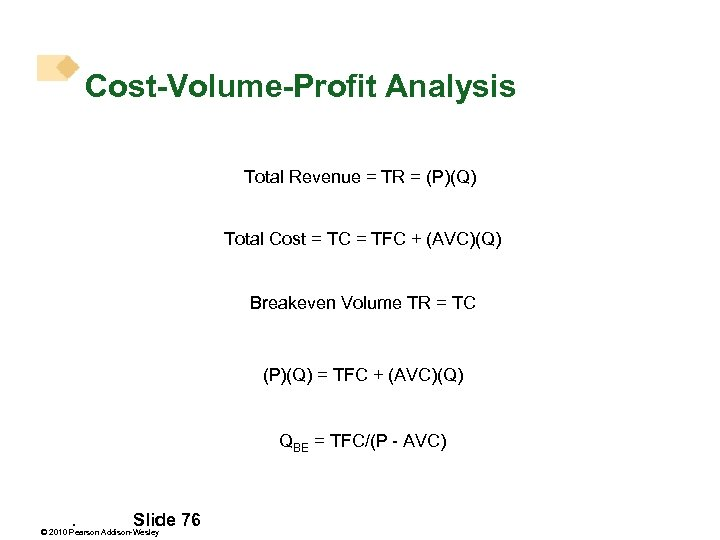Cost-Volume-Profit Analysis Total Revenue = TR = (P)(Q) Total Cost = TC = TFC + (AVC)(Q) Breakeven Volume TR = TC (P)(Q) = TFC + (AVC)(Q) QBE = TFC/(P - AVC) . Slide 76 © 2010 Pearson Addison-Wesley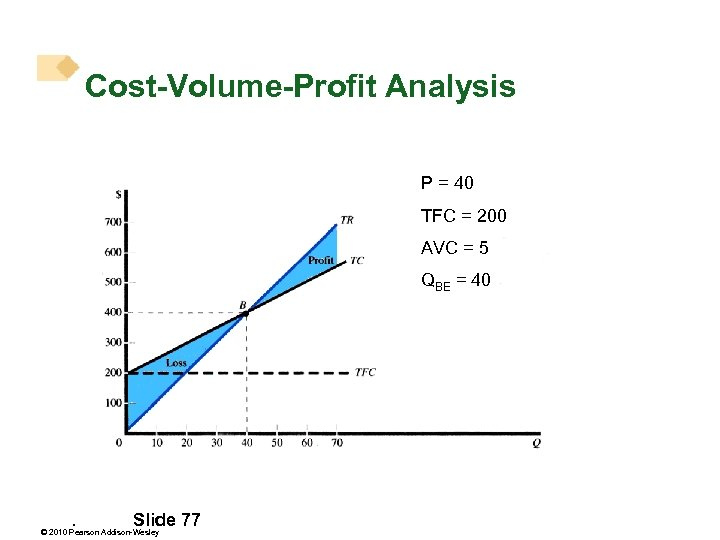Cost-Volume-Profit Analysis P = 40 TFC = 200 AVC = 5 QBE = 40 . Slide 77 © 2010 Pearson Addison-Wesley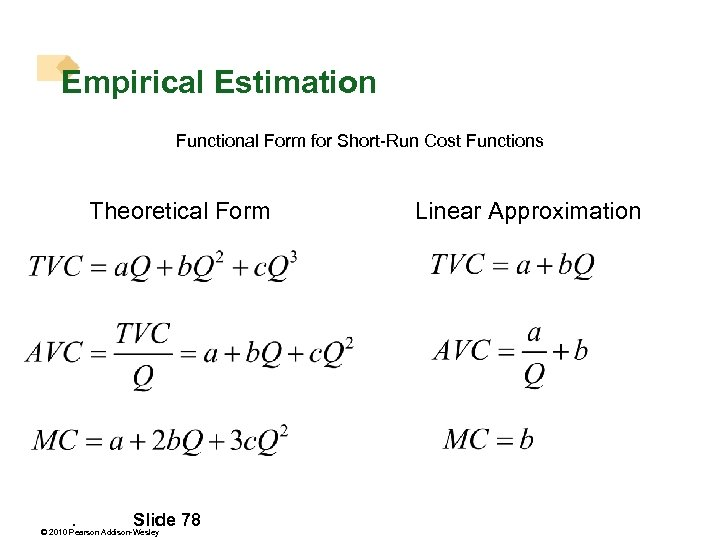Empirical Estimation Functional Form for Short-Run Cost Functions Theoretical Form . Slide 78 © 2010 Pearson Addison-Wesley Linear Approximation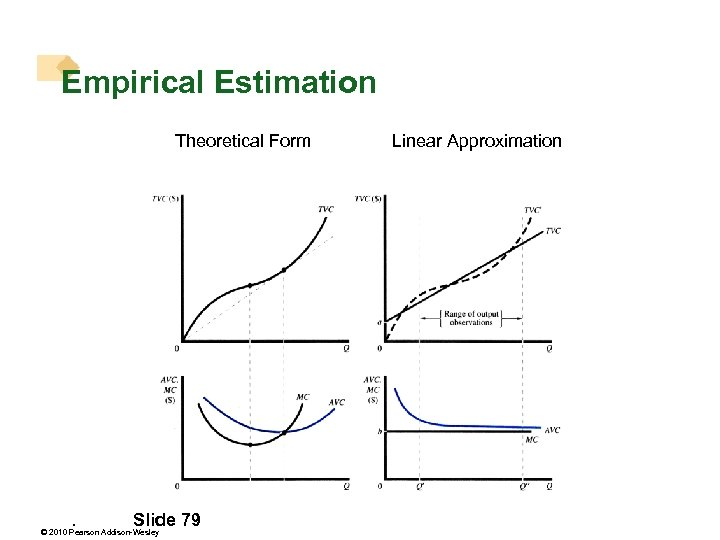Empirical Estimation Theoretical Form . Slide 79 © 2010 Pearson Addison-Wesley Linear Approximation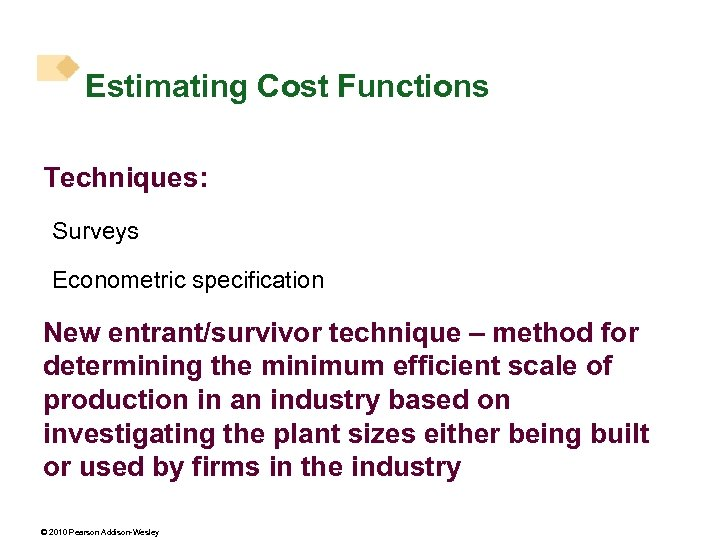Estimating Cost Functions Techniques: Surveys Econometric specification New entrant/survivor technique – method for determining the minimum efficient scale of production in an industry based on investigating the plant sizes either being built or used by firms in the industry © 2010 Pearson Addison-Wesley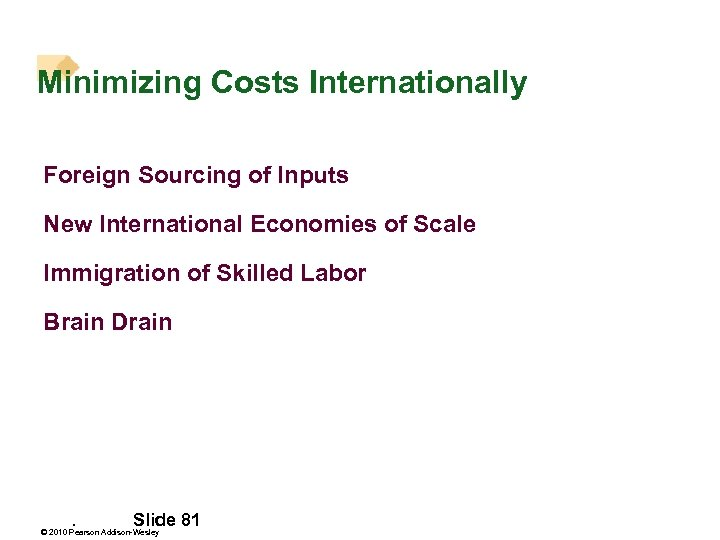Minimizing Costs Internationally Foreign Sourcing of Inputs New International Economies of Scale Immigration of Skilled Labor Brain Drain . Slide 81 © 2010 Pearson Addison-Wesley